Last updated: 2021-06-04

Checks: 7 0

Knit directory: methyl-geneset-testing/

This reproducible R Markdown analysis was created with workflowr (version 1.6.2). The Checks tab describes the reproducibility checks that were applied when the results were created. The Past versions tab lists the development history.

Great! Since the R Markdown file has been committed to the Git repository, you know the exact version of the code that produced these results.

Great job! The global environment was empty. Objects defined in the global environment can affect the analysis in your R Markdown file in unknown ways. For reproduciblity it's best to always run the code in an empty environment.

The command set.seed(20200302) was run prior to running the code in the R Markdown file. Setting a seed ensures that any results that rely on randomness, e.g. subsampling or permutations, are reproducible.

Great job! Recording the operating system, R version, and package versions is critical for reproducibility.

Nice! There were no cached chunks for this analysis, so you can be confident that you successfully produced the results during this run.

Great job! Using relative paths to the files within your workflowr project makes it easier to run your code on other machines.

Great! You are using Git for version control. Tracking code development and connecting the code version to the results is critical for reproducibility.

The results in this page were generated with repository version 19e3838. See the Past versions tab to see a history of the changes made to the R Markdown and HTML files.

Note that you need to be careful to ensure that all relevant files for the analysis have been committed to Git prior to generating the results (you can use wflow_publish or wflow_git_commit). workflowr only checks the R Markdown file, but you know if there are other scripts or data files that it depends on. Below is the status of the Git repository when the results were generated:


Ignored files:
Ignored:    .DS_Store
Ignored:    .Rhistory
Ignored:    .Rproj.user/
Ignored:    analysis/figures.nb.html
Ignored:    code/.DS_Store
Ignored:    code/.Rhistory
Ignored:    code/.job/
Ignored:    code/old/
Ignored:    data/.DS_Store
Ignored:    data/annotations/
Ignored:    data/cache-intermediates/
Ignored:    data/cache-region/
Ignored:    data/cache-rnaseq/
Ignored:    data/cache-runtime/
Ignored:    data/datasets/.DS_Store
Ignored:    data/datasets/GSE110554-data.RData
Ignored:    data/datasets/GSE120854/
Ignored:    data/datasets/GSE120854_RAW.tar
Ignored:    data/datasets/GSE135446-data.RData
Ignored:    data/datasets/GSE135446/
Ignored:    data/datasets/GSE135446_RAW.tar
Ignored:    data/datasets/GSE45459-data.RData
Ignored:    data/datasets/GSE45459_Matrix_signal_intensities.txt
Ignored:    data/datasets/GSE45460/
Ignored:    data/datasets/GSE45460_RAW.tar
Ignored:    data/datasets/GSE95460_RAW.tar
Ignored:    data/datasets/GSE95460_RAW/
Ignored:    data/datasets/GSE95462-data.RData
Ignored:    data/datasets/GSE95462/
Ignored:    data/datasets/GSE95462_RAW/
Ignored:    data/datasets/SRP100803/
Ignored:    data/datasets/SRP125125/.DS_Store
Ignored:    data/datasets/SRP125125/SRR6298*/
Ignored:    data/datasets/SRP125125/SRR_Acc_List.txt
Ignored:    data/datasets/SRP125125/SRR_Acc_List_Full.txt
Ignored:    data/datasets/SRP125125/SraRunTable.txt
Ignored:    data/datasets/SRP125125/multiqc_data/
Ignored:    data/datasets/SRP125125/multiqc_report.html
Ignored:    data/datasets/SRP125125/quants/
Ignored:    data/datasets/SRP166862/
Ignored:    data/datasets/SRP217468/
Ignored:    data/datasets/TCGA.BRCA.rds
Ignored:    data/datasets/TCGA.KIRC.rds
Ignored:    data/misc/
Ignored:    output/--exclude
Ignored:    output/.DS_Store
Ignored:    output/FDR-analysis/
Ignored:    output/compare-methods/
Ignored:    output/figures/
Ignored:    output/methylgsa-params/
Ignored:    output/outputs-1.tar.gz
Ignored:    output/outputs.tar.gz
Ignored:    output/random-cpg-sims/

Untracked files:
Untracked:  analysis/old/



Note that any generated files, e.g. HTML, png, CSS, etc., are not included in this status report because it is ok for generated content to have uncommitted changes.

These are the previous versions of the repository in which changes were made to the R Markdown (analysis/05_compareMethodsBcells.Rmd) and HTML (docs/05_compareMethodsBcells.html) files. If you've configured a remote Git repository (see ?wflow_git_remote), click on the hyperlinks in the table below to view the files as they were in that past version.

File Version Author Date Message
Rmd 19e3838 JovMaksimovic 2021-06-04 wflow_publish("analysis/05_compareMethodsBcells.Rmd")
html 0f8d628 JovMaksimovic 2021-04-20 Build site.
Rmd 9d23572 JovMaksimovic 2021-04-20 wflow_publish(rownames(stat$$status)[stat$$status$modified == TRUE]) Rmd 7a5cd6a JovMaksimovic 2021-04-19 Updated xlims for bubble plot axes to display all points. html 4c57176 JovMaksimovic 2021-04-13 Build site. Rmd 2a8ac36 JovMaksimovic 2021-04-13 Changed RNAseq to Array for B-cell data analyses Rmd 697c330 JovMaksimovic 2021-04-12 Updated figure numbering and labels Rmd 5e34ed3 JovMaksimovic 2021-04-12 Figure updates html 17cc1c9 JovMaksimovic 2021-04-06 Build site. Rmd 58c95d6 JovMaksimovic 2021-04-06 wflow_publish(c("analysis/index.Rmd", "analysis/04_expressionGenesetsBcells.Rmd", html 9029ba4 Jovana Maksimovic 2021-04-02 Build site. Rmd c62fb2c Jovana Maksimovic 2021-04-02 wflow_publish("analysis/05_compareMethodsBcells.Rmd") Rmd 18ac8e3 Jovana Maksimovic 2021-04-02 Methods comparison using B-cell development data. Rmd 73c6790 JovMaksimovic 2021-04-02 Analysis of B-cell data library(here) library(minfi) library(paletteer) library(limma) library(BiocParallel) library(reshape2) library(gridExtra) library(missMethyl) library(ggplot2) library(glue) library(grid) library(tidyverse) library(rbin) library(patchwork) library(ChAMPdata) library(lemon) source(here("code/utility.R")) # Load data We are using publicly available 450K data GSE45459 generated from developing human B-cells. Specifically, the GSE45459_Matrix_signal_intensities.txt.gz file should be downloaded and placed in the data\datasets directory and unzipped using gunzip GSE45459_Matrix_signal_intensities.txt.gz. The data is then normalised and filtered (bad probes, multi-mapping probes, SNP probes, sex chromosomes). # load data dataFile <- here("data/datasets/GSE45459-data.RData") if(file.exists(dataFile)){ # load processed data and sample information load(dataFile) } else { raw <- read.delim(here("data/datasets/GSE45459_Matrix_signal_intensities.txt"), row.names = "ID_REF") M <- raw[,grepl("Methylated", colnames(raw))] U <- raw[,grepl("Unmethylated", colnames(raw))] detP <- raw[,grepl("Pval", colnames(raw))] targets <- as.data.frame(strsplit2(colnames(detP),"_")[,1:2]) colnames(targets) <- c("ID","Stage") mSet <- MethylSet(Meth = as.matrix(M), Unmeth = as.matrix(U), annotation = c(array = "IlluminaHumanMethylation450k", annotation = "ilmn12.hg19")) colnames(mSet) <- targets$ID
grRaw <- minfi::mapToGenome(mSet)

#normalise
normGr <- preprocessQuantile(grRaw)
#filter
fltGr <- filterQual(normGr = normGr, detP = detP)
fltGr <- filterProbes(fltGr)

save(detP, normGr, fltGr, targets, file = dataFile)

}

## QC plots

# plot mean detection p-values across all samples
dat <- tibble::tibble(mean = colMeans(detP), group = targets$Stage, sample = targets$ID)
ggplot(dat, aes(y = mean, x = sample, fill = group)) +
geom_bar(stat = "identity") +
labs(fill = "Stage",
x = "Sample",
y = "Mean detection p-value") +
theme(axis.text.x = element_text(angle = 90, vjust = 0.5))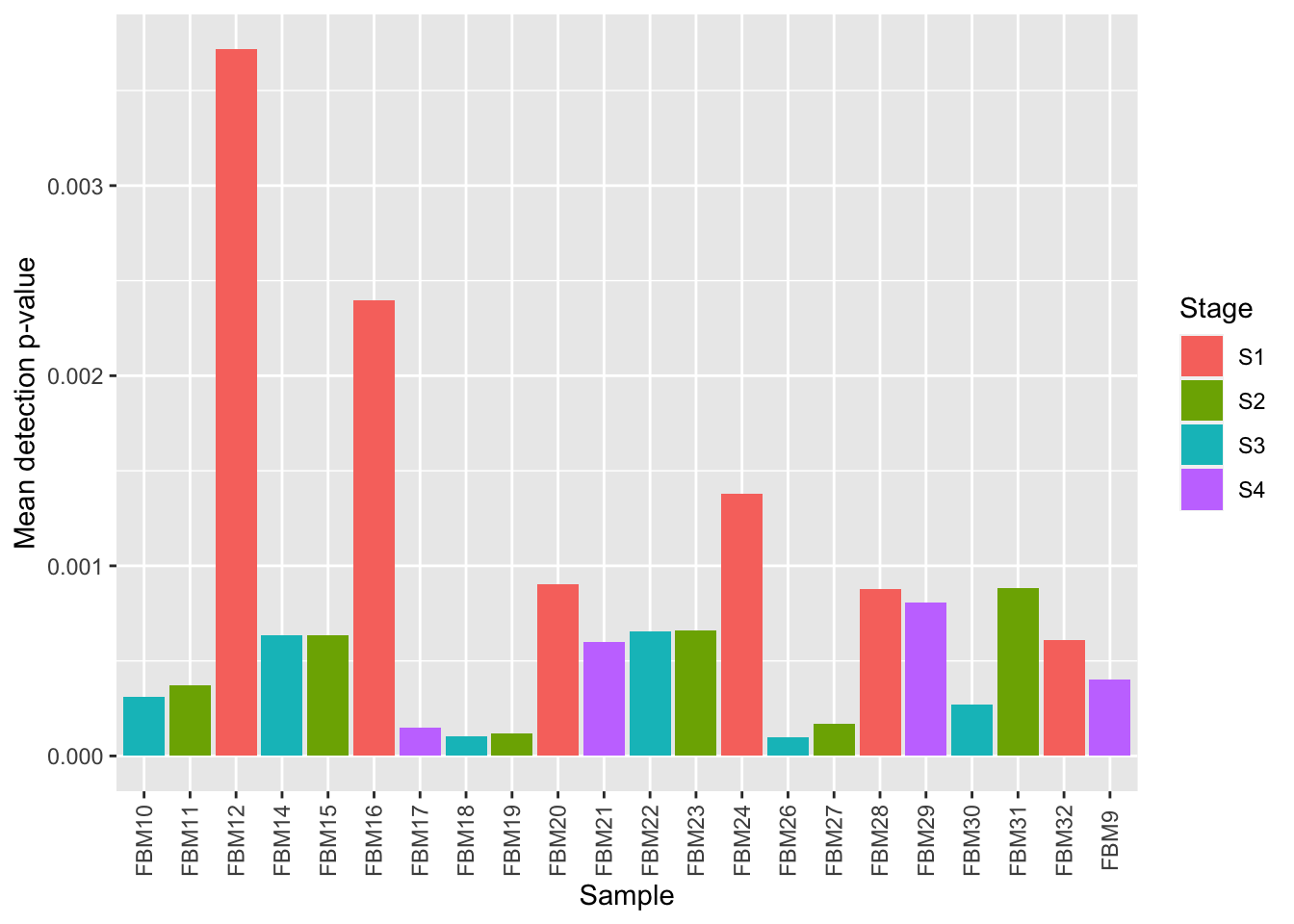Version Author Date
17cc1c9 JovMaksimovic 2021-04-06
9029ba4 Jovana Maksimovic 2021-04-02
# plot normalised beta value distribution
bVals <- getBeta(fltGr)
dat <- as_tibble(reshape2::melt(bVals))
colnames(dat) <- c("cpg", "ID", "beta")
dat$group <- rep(targets$Stage, each = nrow(bVals))

p <- ggplot(dat, aes(x = beta, colour = group, group = ID)) +
geom_density(size=0.5, alpha=0.5) +
labs(color = "Stage", x = "Beta value", y = "Density")

p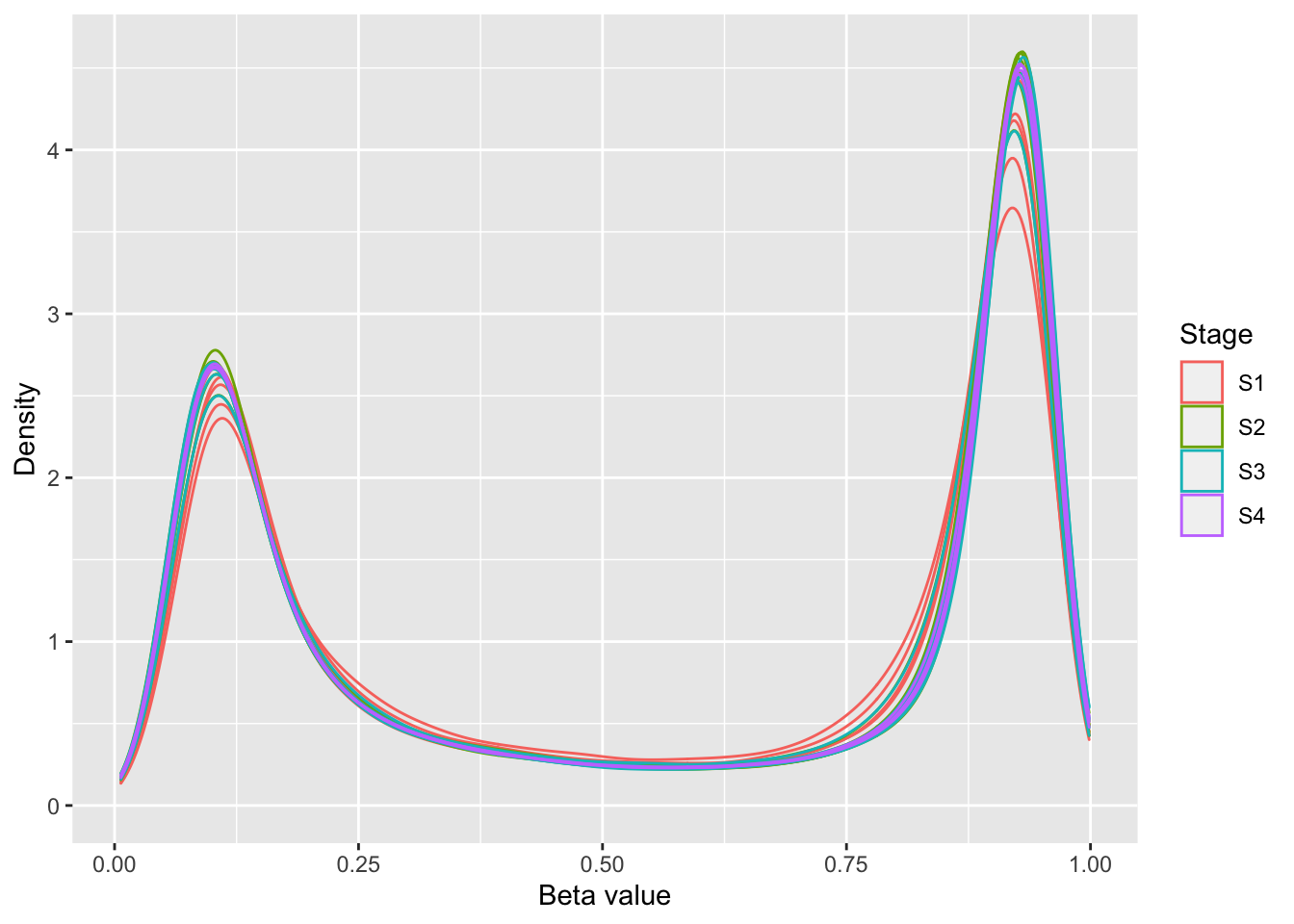Version Author Date
17cc1c9 JovMaksimovic 2021-04-06
9029ba4 Jovana Maksimovic 2021-04-02
dims <- list(c(1,2), c(1,3), c(2,3), c(3,4))
p <- vector("list", length(dims))

for(i in 1:length(dims)){
tmp <- plotMDS(getM(fltGr), top=1000,
gene.selection="common", plot = FALSE,
dim.plot = dims[[i]])

dat <- data.frame(x = tmp$x, y = tmp$y, group = targets$Stage) p[[i]] <- ggplot(dat, aes(x = x, y = y, colour = group)) + geom_point() + labs(colour = "Stage", x = glue("PC {tmp$dim.plot}"),
y = glue("PC {tmp$dim.plot}")) } (p[] | p[]) / (p[] | p[]) + plot_layout(guides = "collect")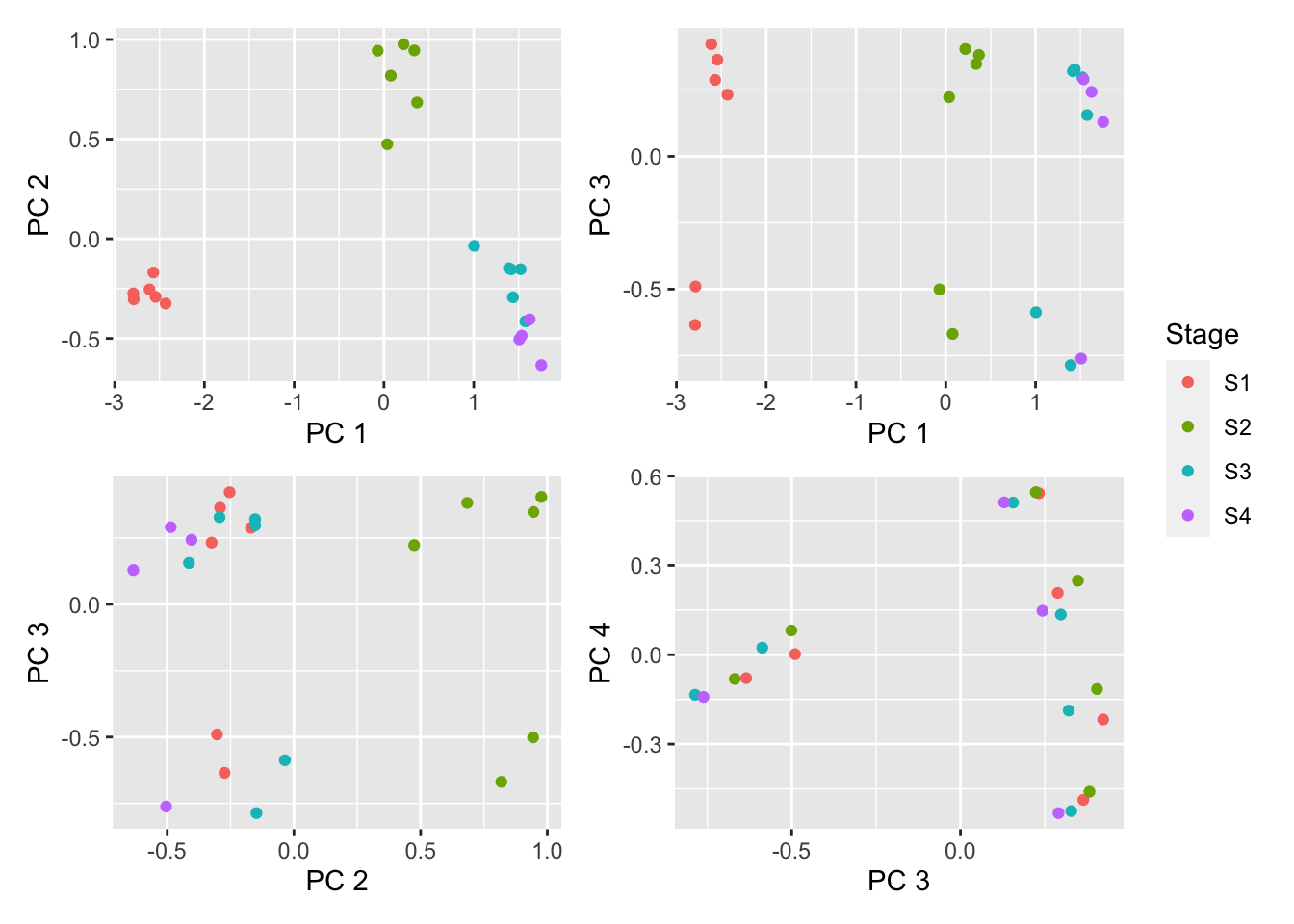Version Author Date 17cc1c9 JovMaksimovic 2021-04-06 9029ba4 Jovana Maksimovic 2021-04-02 Save figure for use in manuscript. outDir <- here::here("output/figures") if (!dir.exists(outDir)) dir.create(outDir) fig <- here("output/figures/SFig-8A.rds") saveRDS(p[], fig, compress = FALSE) ## Statistical analysis Compare Stage 1 and Stage 2 of pre B-cell development. Consider results significant at FDR < 0.05 and delta beta > 10% (~ lfc = 0.5). mVals <- getM(fltGr) bVals <- getBeta(fltGr) Stage <- factor(targets$Stage)
design <- model.matrix(~0+Stage)

fit <- lmFit(mVals, design)
cont.matrix <- makeContrasts(S1vS2=StageS1-StageS2,
levels=design)
fit2 <- contrasts.fit(fit, cont.matrix)
fit2 <- eBayes(fit2, robust=TRUE, trend=TRUE)
tfit <- treat(fit2, lfc = 0.5)
pval <- 0.05
fitSum <- summary(decideTests(tfit, p.value = pval))
fitSum
        S1vS2
Down     1083
NotSig 366973
Up       2065
bDat <- getBiasDat(rownames(topTreat(tfit, coef = 1, num = 5000,
p.value = 0.05)),
array.type = "450K")
All input CpGs are used for testing.
p <- ggplot(bDat, aes(x = avgbias, y = propDM)) +
geom_point(shape = 1, size = 2) +
geom_smooth() +
labs(x = "No. CpGs per gene (binned)",
y = "Prop. differential methylation") +
theme_minimal() +
theme(panel.grid = element_blank(),
axis.line = element_line(colour = "black"))
p
geom_smooth() using method = 'loess' and formula 'y ~ x'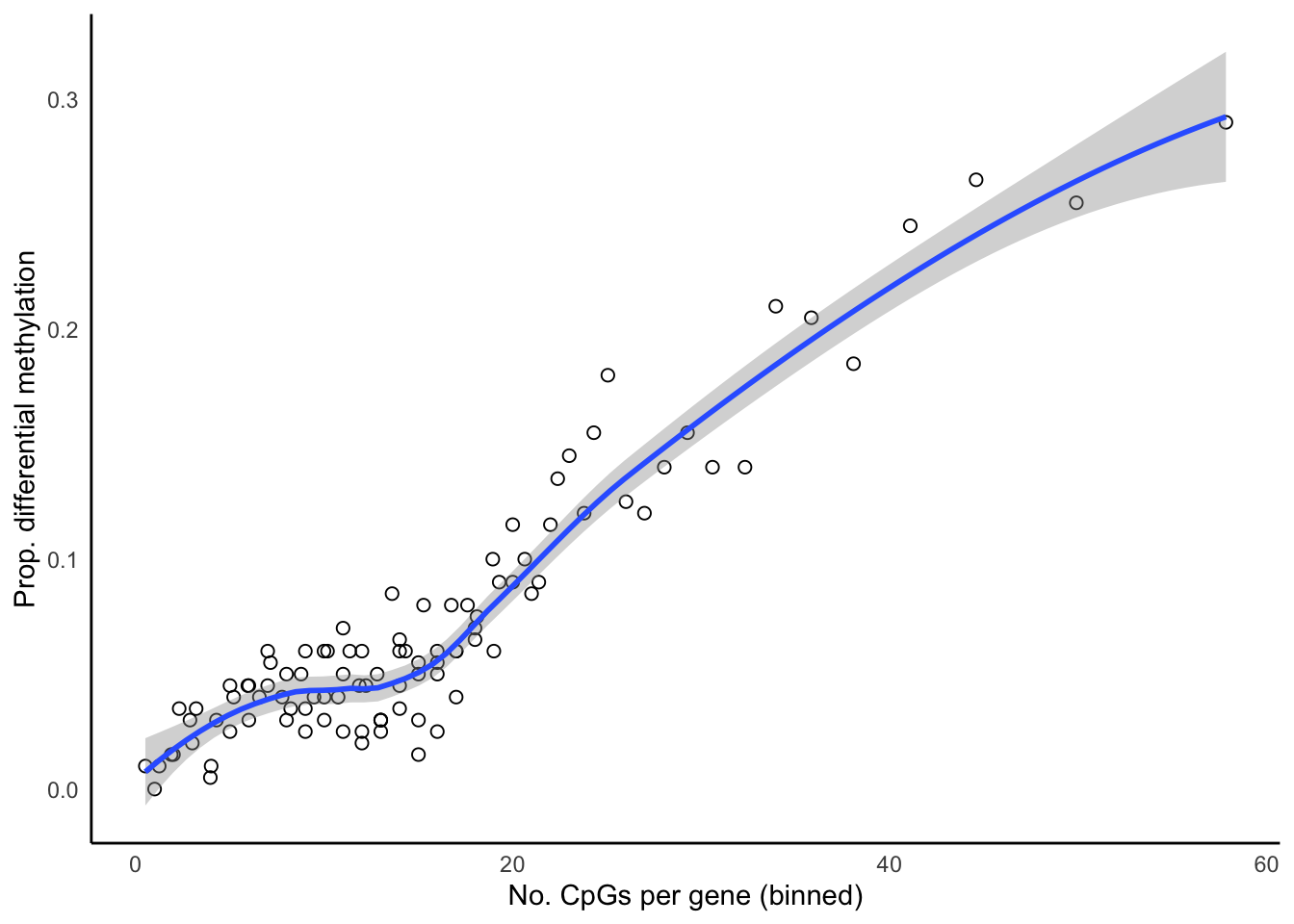Version Author Date
17cc1c9 JovMaksimovic 2021-04-06
9029ba4 Jovana Maksimovic 2021-04-02

Examine only the independent contrasts.

fitSum %>%
data.frame %>%
rename_with(.fn = ~ c("Direction", "Comparison", "Value")) %>%
filter(Direction != "NotSig") -> dat

p <- ggplot(dat, aes(x = Comparison, y = Value, fill = Direction)) +
geom_bar(stat = "identity", position = "dodge") +
labs(x = "Comparison", y = glue("No. DM CpGs (FDR < {pval})"),
fill = "Direction") +
scale_fill_brewer(palette = "Set1", direction = -1)
p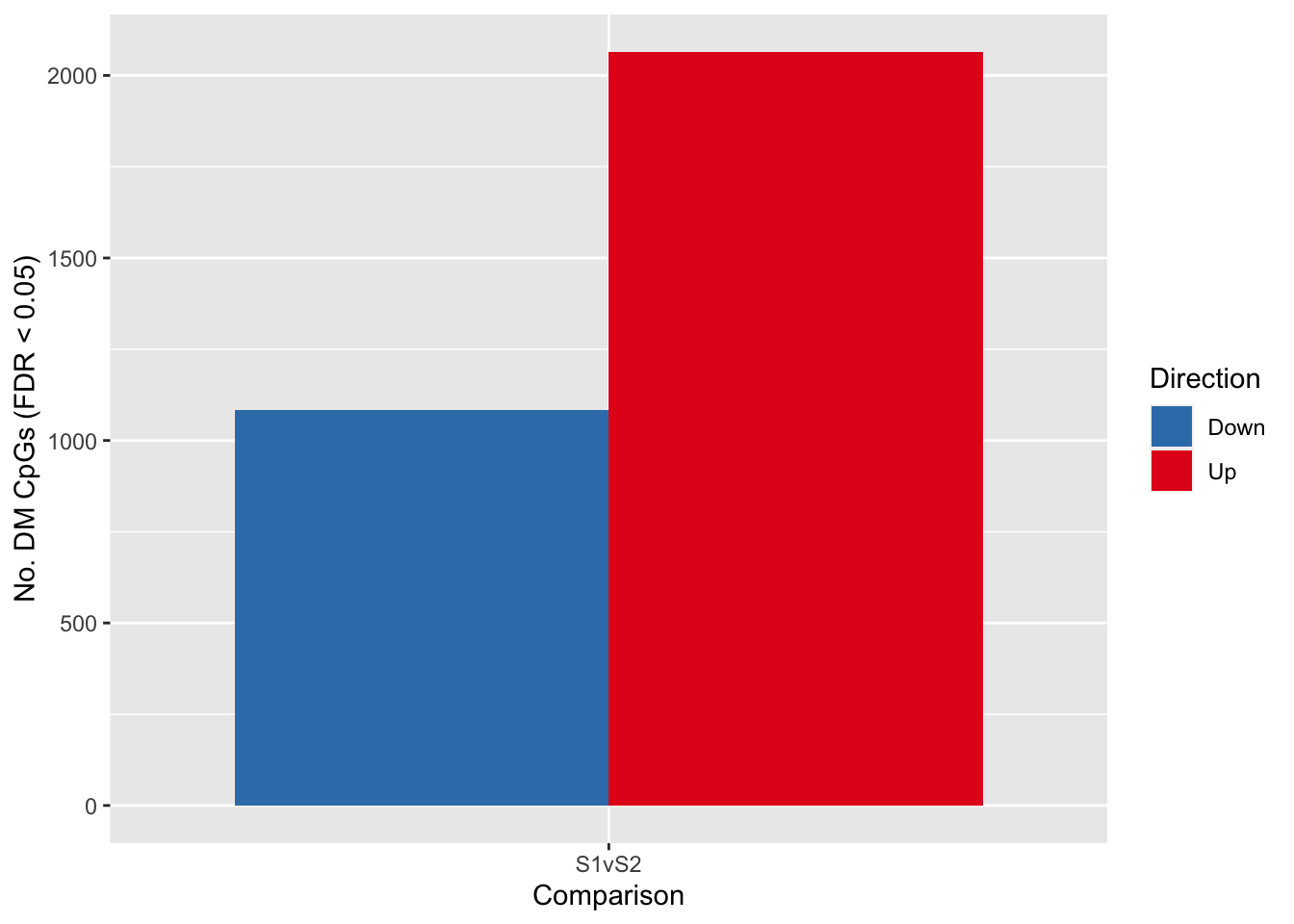Version Author Date
4c57176 JovMaksimovic 2021-04-13

Save figure for use in manuscript.

fig <- here("output/figures/SFig-8B.rds")
saveRDS(p, fig, compress = FALSE)

# Compare performance of different gene set testing methods

We compare the performance of the following gene set testing methods available for methylation arrays: a standard hypergeometric test (HGT), GOmeth from the missMethyl package, methylglm (mGLM), methylRRA-ORA (mRRA (ORA)) and methylRRA-GSEA (mRRA (GSEA)) from the methylGSA package and ebGSEA from GitHub at https://github.com/aet21/ebGSEA.

We perform gene set testing on the results of all three blood cell type comparisons: B-cells vs. NK cells, CD4 T-cells vs. CD8 T-cells and monocytes vs. neutrophils, using all of the different gene set testing methods.

Gene set testing is performed for each comparison using GO categories, KEGG pathways and the BROAD MSigDB gene sets for the following methods: HGT, GOmeth, mGLM, mRRA (ORA), mRRA (GSEA), ebGSEA (WT) and ebGSEA (KPMT).

As the methylGSA methods do not work well with sets that only contain very few genes or very many genes, we only test sets with at least 5 genes and at most 5000 genes.

Firstly, the results of the statistical analysis of the three blood cell comparisons are saved as an RDS object.

minsize <- 5
maxsize <- 5000

outDir <- here::here("data/cache-intermediates/BCELLS")
if (!dir.exists(outDir)) dir.create(outDir)

outFile <- here("data/cache-intermediates/bcells.contrasts.rds")

if(!file.exists(outFile)){
obj <- NULL
obj$tfit <- tfit obj$maxsize <- maxsize
obj$minsize <- minsize obj$mVals <- mVals
obj$targets <- targets saveRDS(obj, file = outFile) }  As some of the methods take a considerable amount of time to perform the gene set testing analysis, we have created several scripts in order to run the analyses in parallel on a HPC. The code used to run all the gene set testing analyses using the different methods can be found in the code/compare-methods directory. It consists of four scripts: genRunMethodJob.R, runebGSEA.R, runMethylGSA.R and runMissMethyl.R. The genRunMethodJob.R script creates and submits Slurm job scripts that run the runebGSEA.R, runMethylGSA.R and runMissMethyl.R scripts, for each gene set type, in parallel, on a HPC. The results of each job are saved as an RDS file named {package}.{set}.rds in the output/compare-methods/BCELLS directory. Once all analysis jobs are complete, all of the subsequent analyses in this document can be executed ## Load output for all methods The results of all the gene set testing analyses, using all the different methods, for the different types of gene sets, are loaded into a list of data.frames. All of the data.frames in the list are then concatenated into a tibble for downstream analysis and plotting. inFiles <- list.files(here("output/compare-methods/BCELLS"), pattern = "rds", full.names = TRUE) res <- lapply(inFiles, function(file){ readRDS(file) }) dat <- as_tibble(dplyr::bind_rows(res)) ## Explore analysis results ### GO categories Examine the performance of the different methods when gene set testing was performed on GO categories. ann <- loadAnnotation("450k") flatAnn <- loadFlatAnnotation(ann) cpgEgGo <- cpgsEgGoFreqs(flatAnn) cpgEgGo %>% group_by(GO) %>% summarise(med = median(Freq)) -> medCpgEgGo In order to examine whether the probe-number bias influenced the significantly enriched GO categories for the different methods, we split the GO categories into bins based on the median number of CpGs per gene per GO category. We then calculated the proportion of significantly enriched GO categories in each bin for each of the three comparisons. Apart from mRRA (GSEA), none of the methods showed a trend related to siez of GO categories. dat %>% filter(set == "GO") %>% filter(sub %in% c("n","p1")) %>% mutate(method = unname(dict[method])) %>% inner_join(medCpgEgGo, by = c("ID" = "GO")) -> sub bins <- rbin_quantiles(sub, ID, med, bins = 11) sub$bin <- as.factor(findInterval(sub$med, bins$upper_cut))

binLabs <- paste0("<", bins$upper_cut) names(binLabs) <- levels(sub$bin)
binLabs[length(binLabs)] <- gsub("<", "\u2265", binLabs[length(binLabs) - 1])

sub %>% group_by(contrast, method, bin) %>%
summarise(prop = sum(pvalue < 0.05)/n()) -> pdat
summarise() has grouped output by 'contrast', 'method'. You can override using the .groups argument.
p <- ggplot(pdat, aes(x = as.numeric(bin), y = prop, color = method)) +
geom_line() +
facet_wrap(vars(contrast), ncol = 3) +
scale_x_continuous(breaks = as.numeric(levels(pdat$bin)), labels = binLabs) + theme(axis.text.x = element_text(angle=45, hjust = 1, vjust = 1, size = 7), legend.position = "bottom") + labs(x = "Med. No. CpGs per Gene per GO Cat. (binned)", y = "Prop. GO Cat. with p-value < 0.05", colour = "Method") + scale_color_manual(values = methodCols) p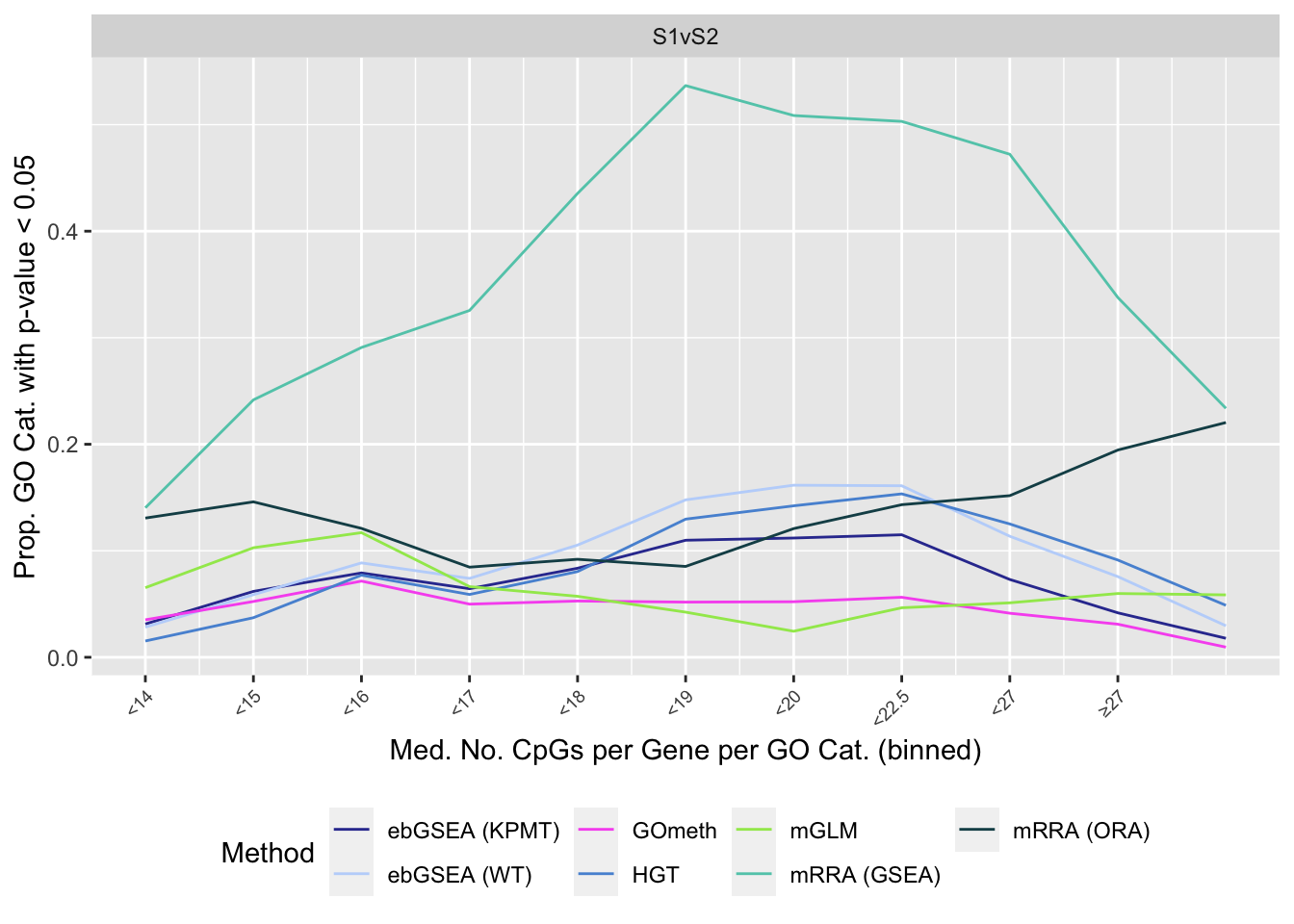Version Author Date 4c57176 JovMaksimovic 2021-04-13 We also examine results when top ranked 100 GO terms from gene expression array analysis of the same B-cell development stage comparisons is used as "truth". immuneGO <- unique(read.csv(here("data/genesets/GO-immune-system-process.txt"), stringsAsFactors = FALSE, header = FALSE, col.names = "GOID")) rnaseqGO <- readRDS(here("data/cache-rnaseq/RNAseq-GSE45460-GO.rds")) rnaseqGO %>% group_by(contrast) %>% mutate(rank = 1:n()) %>% filter(rank <= 100) -> topGOSets p <- vector("list", length(unique(dat$contrast)))

for(i in 1:length(unique(dat$contrast))){ cont <- sort(unique(dat$contrast))[i]

dat %>% filter(set == "GO") %>%
filter(sub %in% c("n","p1")) %>%
filter(contrast == cont) %>%
mutate(method = unname(dict[method])) %>%
arrange(method, pvalue) %>%
group_by(method) %>%
mutate(rank = 1:n()) %>%
filter(rank <= 100) %>%
mutate(csum = cumsum(ID %in% immuneGO$GOID)) %>% mutate(truth = "ISP Terms") -> immuneSum dat %>% filter(set == "GO") %>% filter(sub %in% c("n","p1")) %>% filter(contrast == cont) %>% mutate(method = unname(dict[method])) %>% arrange(method, pvalue) %>% group_by(method) %>% mutate(rank = 1:n()) %>% filter(rank <= 100) %>% mutate(csum = cumsum(ID %in% topGOSets$ID[topGOSets$contrast %in% contrast])) %>% mutate(truth = "Array Terms") -> rnaseqSum truthSum <- bind_rows(immuneSum, rnaseqSum) p[[i]] <- ggplot(truthSum, aes(x = rank, y = csum, colour = method)) + geom_line() + facet_wrap(vars(truth), ncol = 2) + geom_vline(xintercept = 10, linetype = "dotted") + labs(colour = "Method", x = "Rank", y = "Cumulative no. sets in truth") + theme(legend.position = "bottom") + scale_color_manual(values = methodCols) } p[] + ggtitle(sort(unique(dat$contrast)))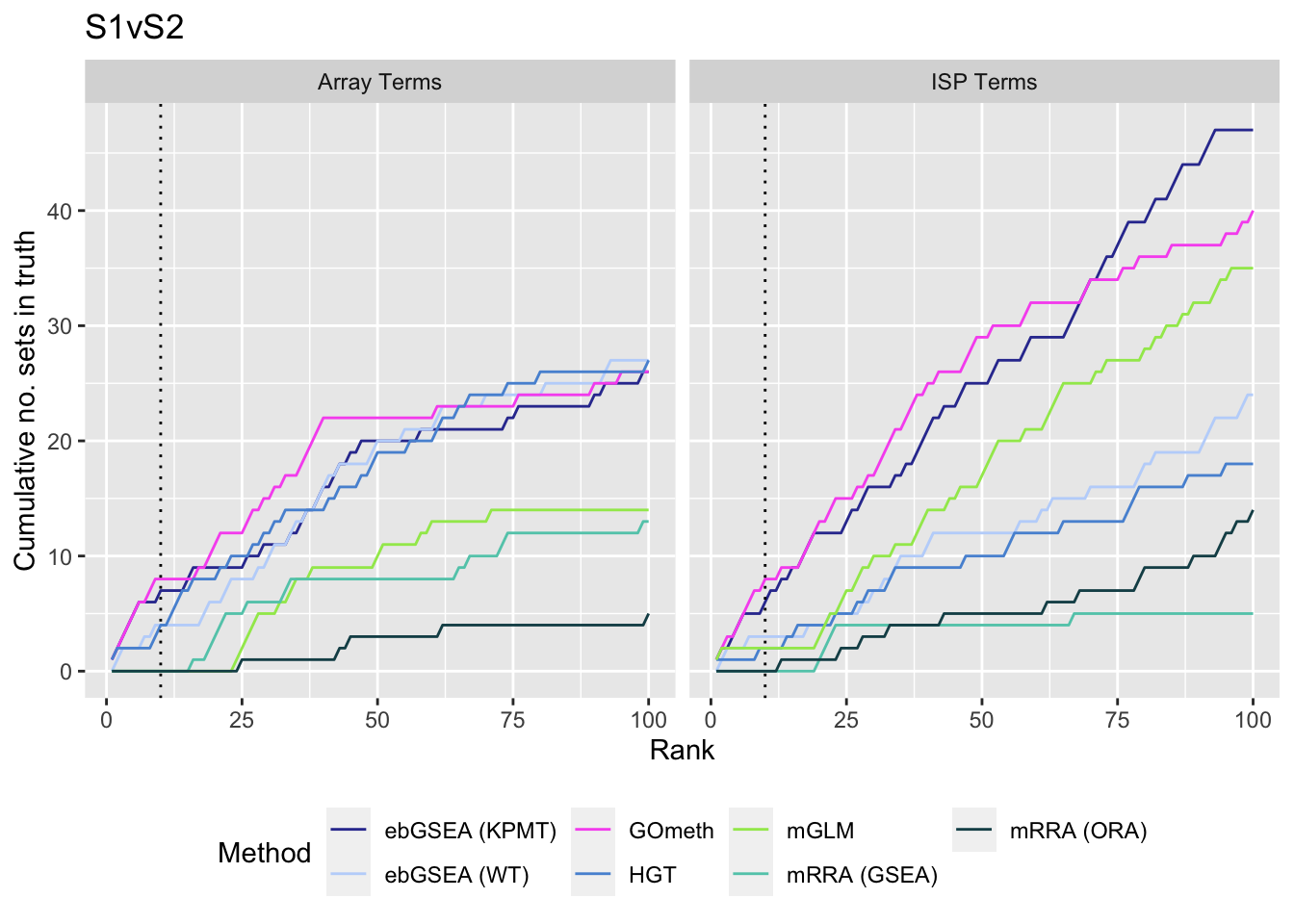Version Author Date
4c57176 JovMaksimovic 2021-04-13
17cc1c9 JovMaksimovic 2021-04-06
9029ba4 Jovana Maksimovic 2021-04-02

Save figure for use in manuscript.

fig <- here("output/figures/SFig-8C.rds")
saveRDS(shift_legend(p[] + theme(plot.title = element_blank()),
pos = "left"),
fig, compress = FALSE)

Examine what the top 10 ranked gene sets are and how many genes they contain, for each method and comparison.

terms <- missMethyl:::.getGO()$idTable nGenes <- rownames_to_column(data.frame(n = sapply(missMethyl:::.getGO()$idList,
length)),
var = "ID")

dat %>% filter(set == "GO") %>%
filter(sub %in% c("n","p1")) %>%
mutate(method = unname(dict[method])) %>%
arrange(contrast, method, pvalue) %>%
group_by(contrast, method) %>%
mutate(FDR = p.adjust(pvalue, method = "BH")) %>%
mutate(rank = 1:n()) %>%
filter(rank <= 10) %>%
inner_join(terms, by = c("ID" = "GOID")) %>%
inner_join(nGenes) -> sub
Joining, by = "ID"
p <- vector("list", length(unique(sub$contrast))) truthPal <- scales::hue_pal()(4) names(truthPal) <- c("Both", "ISP", "Neither", "Array") for(i in 1:length(p)){ cont <- sort(unique(sub$contrast))[i]
sub %>% filter(contrast == cont) %>%
arrange(method, -rank) %>%
ungroup() %>%
mutate(idx = as.factor(1:n())) -> tmp

setLabs <- substr(tmp$TERM, 1, 40) names(setLabs) <- tmp$idx

tmp %>% mutate(rna = ID %in% topGOSets$ID[topGOSets$contrast %in% cont],
isp = ID %in% immuneGO$GOID, both = rna + isp, col = ifelse(both == 2, "Both", ifelse(both == 1 & rna == 1, "Array", ifelse(both == 1 & isp == 1, "ISP", "Neither")))) %>% mutate(col = factor(col, levels = c("Both", "ISP", "Array", "Neither"))) -> tmp p[[i]] <- ggplot(tmp, aes(x = -log10(FDR), y = idx, colour = col)) + geom_point(aes(size = n), alpha = 0.7) + scale_size(limits = c(min(sub$n), max(sub$n))) + facet_wrap(vars(method), ncol = 2, scales = "free") + scale_y_discrete(labels = setLabs) + scale_colour_manual(values = truthPal) + labs(y = "", size = "No. genes", colour = "In truth set") + theme(axis.text.y = element_text(size = 6), axis.text.x = element_text(size = 6), legend.box = "horizontal", legend.margin = margin(0, 0, 0, 0, unit = "lines"), panel.spacing.x = unit(1, "lines")) + coord_cartesian(xlim = c(-log10(0.99), -log10(10^-200))) + geom_vline(xintercept = -log10(0.05), linetype = "dashed") + ggtitle(cont) } shift_legend(p[], plot = TRUE, pos = "left")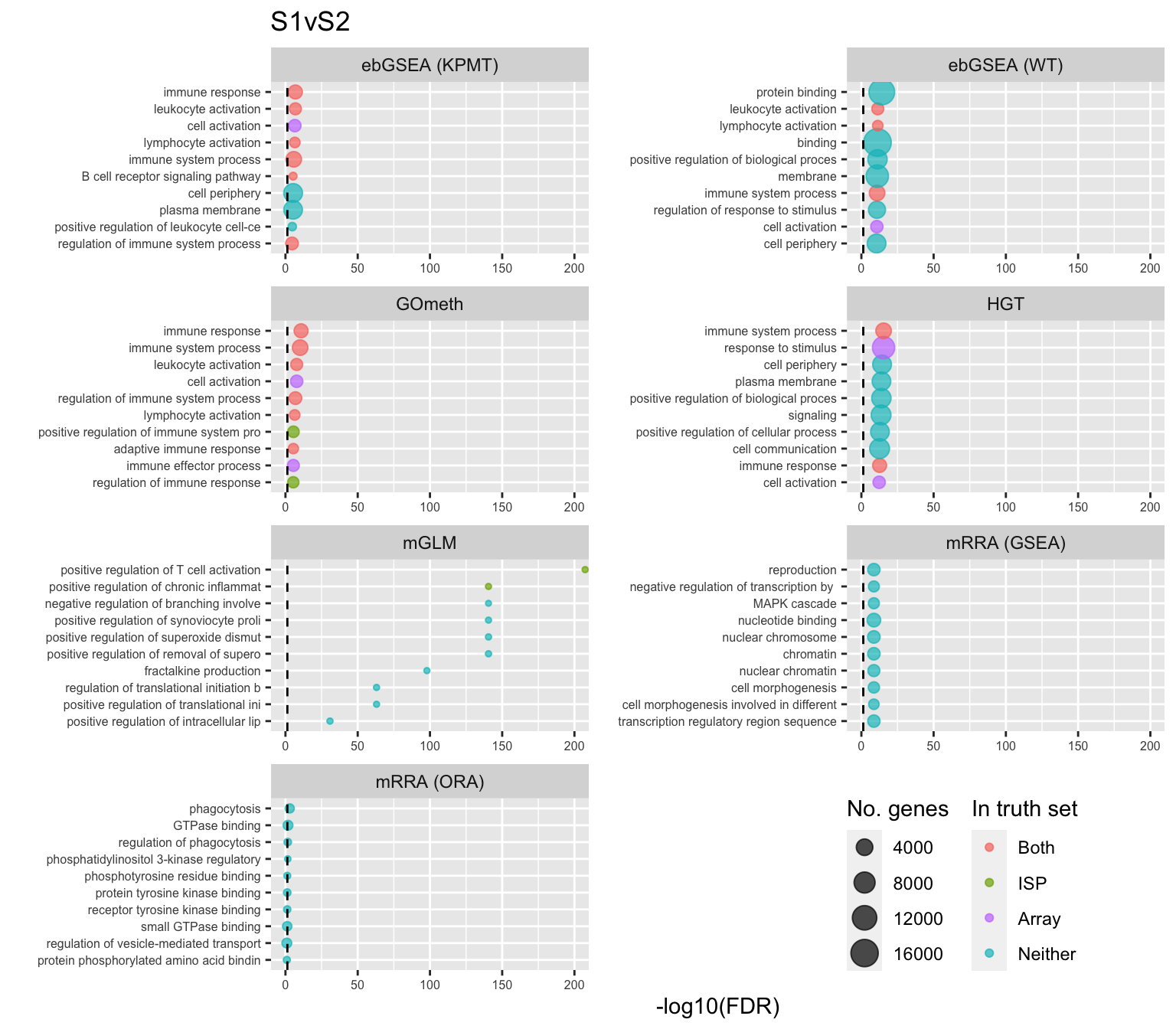Version Author Date 0f8d628 JovMaksimovic 2021-04-20 4c57176 JovMaksimovic 2021-04-13 17cc1c9 JovMaksimovic 2021-04-06 Save figure for use in manuscript. fig <- here("output/figures/SFig-8D.rds") saveRDS(shift_legend(p[] + theme(plot.title = element_blank()), pos = "left"), fig, compress = FALSE) P-value histograms for the different methods for all contrasts on GO categories. dat %>% filter(set == "GO") %>% filter(sub %in% c("n","p1")) %>% mutate(method = unname(dict[method])) -> subDat ggplot(subDat, aes(pvalue, fill = method)) + geom_histogram(binwidth = 0.025) + facet_wrap(vars(method), ncol = 2, nrow = 4) + theme(legend.position = "bottom") + labs(x = "P-value", y = "Frequency", fill = "Method") + scale_fill_manual(values = methodCols)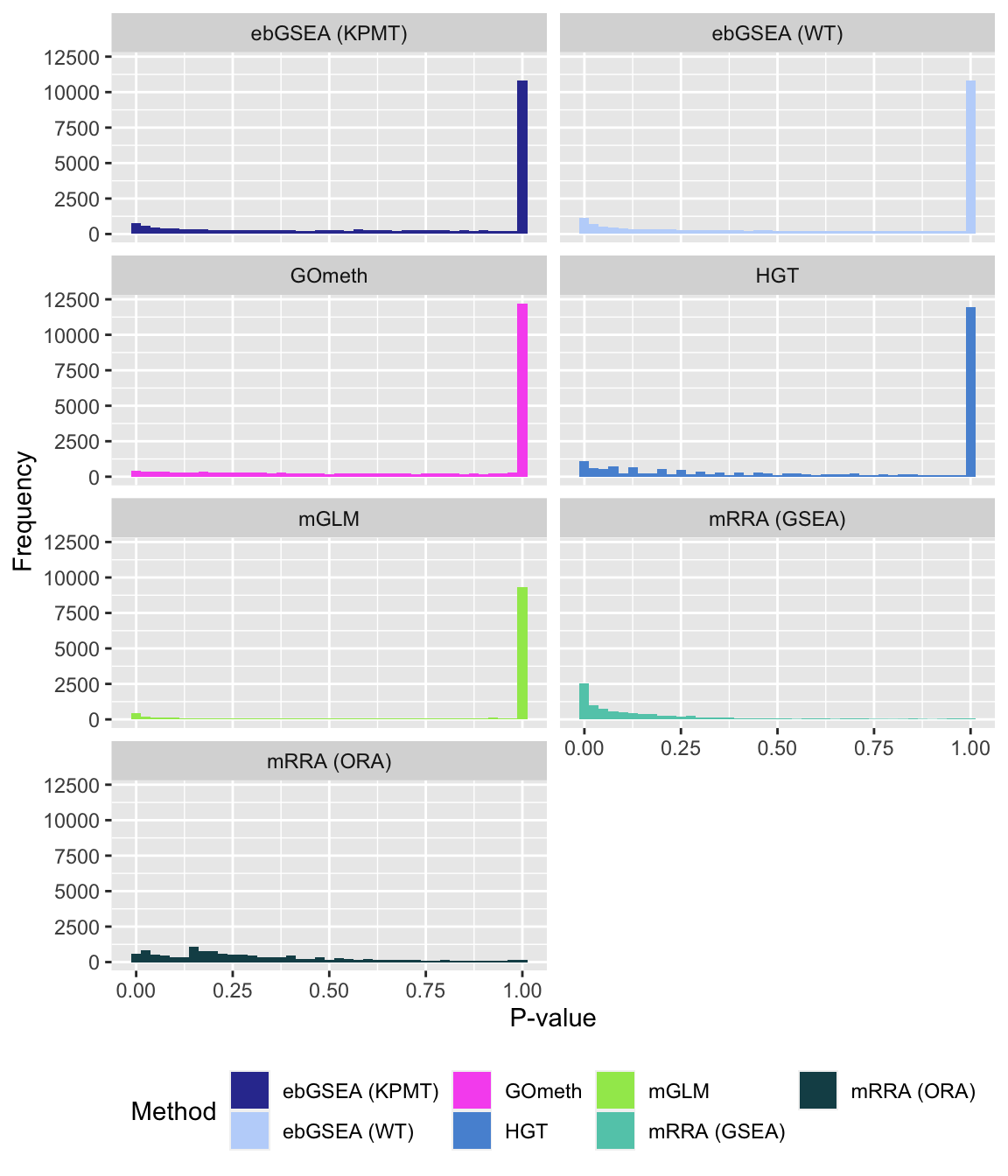Version Author Date 4c57176 JovMaksimovic 2021-04-13 17cc1c9 JovMaksimovic 2021-04-06 #### Compare GOmeth results using different DM CpG significance cutoffs As the results of GOmeth depend on the list of significant CpGs provided as input to the function, we explored the effect of selecting "significant" CpGs in different ways on the gene set testing performance of GOmeth. dat %>% filter(set == "GO") %>% filter(grepl("mmethyl", method)) %>% mutate(method = unname(dict[method])) %>% arrange(contrast, method, pvalue) %>% group_by(contrast, method, sub) %>% mutate(csum = cumsum(ID %in% immuneGO$GOID)) %>%
mutate(rank = 1:n()) %>%
mutate(cut = ifelse(sub == "c1", "Top 5000",
ifelse(sub == "c2", "Top 10000",
ifelse(sub == "p1", "FDR < 0.01", "FDR < 0.05")))) %>%
filter(rank <= 100) -> sub

p <- ggplot(sub, aes(x = rank, y = csum, colour = cut)) +
geom_line() +
facet_wrap(vars(method), ncol=2, nrow = 3, scales = "free") +
geom_vline(xintercept = 10, linetype = "dotted") +
labs(colour = "Sig. select", x = "Rank", y = "Cumulative no. immune sets") +
theme(legend.position = "bottom")
p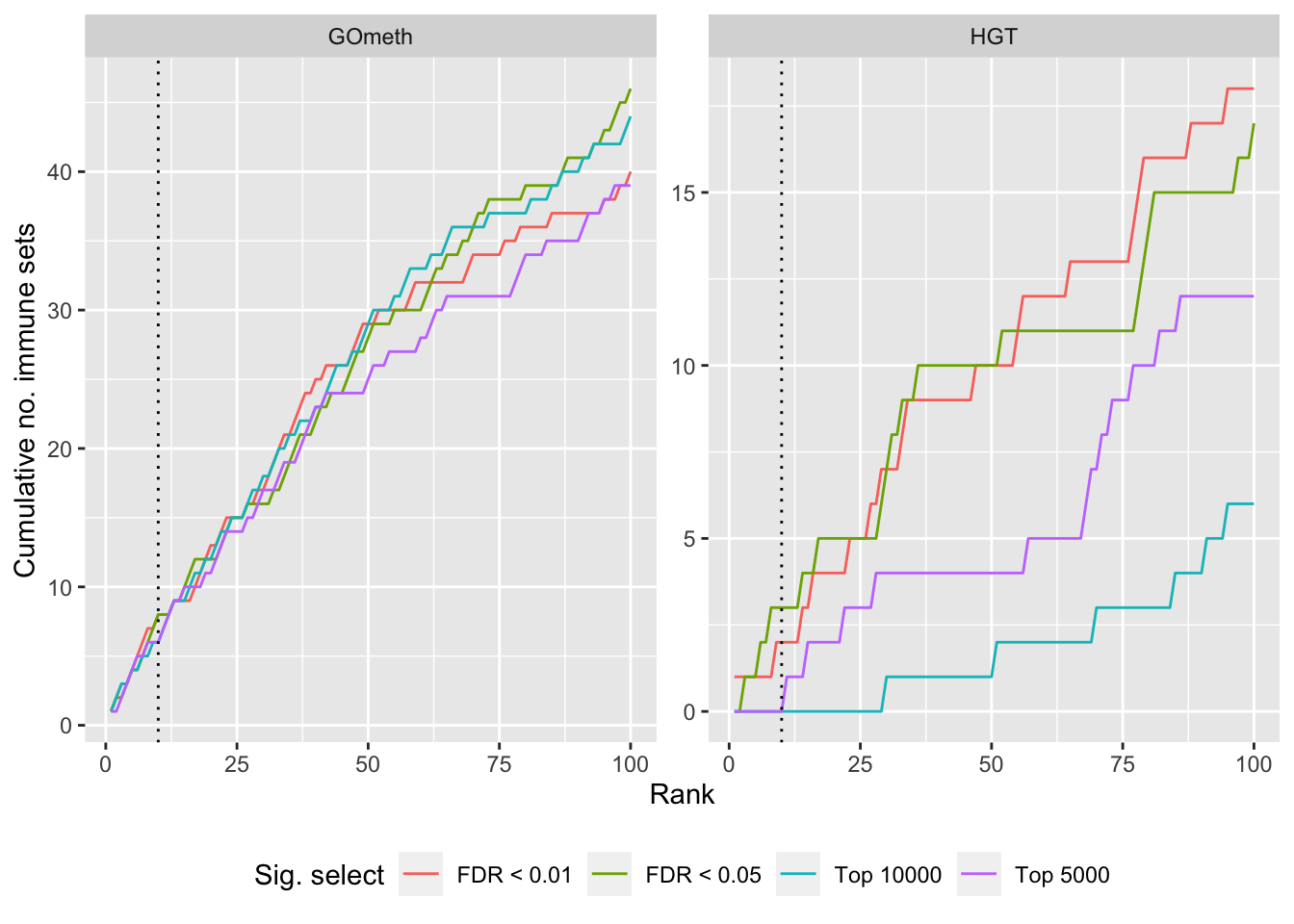Version Author Date
4c57176 JovMaksimovic 2021-04-13
17cc1c9 JovMaksimovic 2021-04-06
9029ba4 Jovana Maksimovic 2021-04-02
dat %>% filter(set == "GO") %>%
filter(grepl("mmethyl", method)) %>%
mutate(method = unname(dict[method])) %>%
arrange(contrast, method, pvalue) %>%
group_by(contrast, method, sub) %>%
mutate(csum = cumsum(ID %in% topGOSets$ID[topGOSets$contrast %in%
contrast])) %>%
mutate(rank = 1:n()) %>%
mutate(cut = ifelse(sub == "c1", "Top 5000",
ifelse(sub == "c2", "Top 10000",
ifelse(sub == "p1", "FDR < 0.01", "FDR < 0.05")))) %>%
filter(rank <= 100) -> sub

p <- ggplot(sub, aes(x = rank, y = csum, colour = cut)) +
geom_line() +
facet_wrap(vars(method), ncol=2, nrow = 3, scales = "free") +
geom_vline(xintercept = 10, linetype = "dotted") +
labs(colour = "Sig. select", x = "Rank",
y = glue("Cumulative no. Array sets")) +
theme(legend.position = "bottom")
p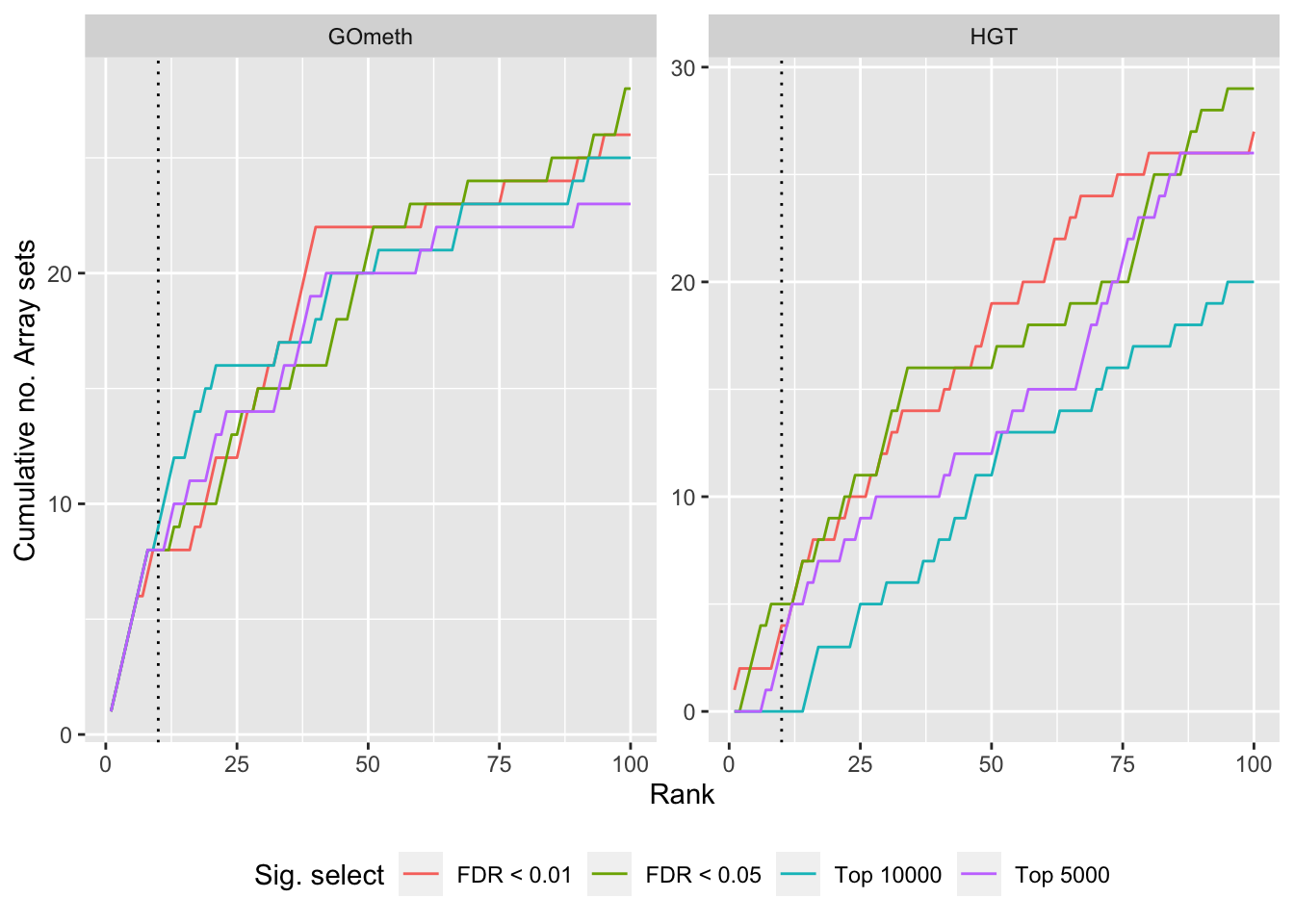Version Author Date
4c57176 JovMaksimovic 2021-04-13
17cc1c9 JovMaksimovic 2021-04-06
9029ba4 Jovana Maksimovic 2021-04-02

### KEGG pathways

Now test KEGG pathways with at least 5 genes and 5000 at most.

Again, as we are comparing immune cells we expect pathways from the following categories to be highly ranked: Immune system, Immune disease, Signal transduction, Signaling molecules and interaction; https://www.genome.jp/kegg/pathway.html.

Examine results when top ranked 100 KEGG pathways from gene expression array analysis of the same B-cell development stage comparisons is used as "truth".

immuneKEGG <- read.csv(here("data/genesets/kegg-immune-related-pathways.csv"),
stringsAsFactors = FALSE, header = FALSE,
col.names = c("ID","pathway"))
immuneKEGG$PID <- paste0("path:hsa0",immuneKEGG$ID)

rnaseqKEGG %>% group_by(contrast) %>%
mutate(rank = 1:n()) %>%
filter(rank <= 100) -> topKEGG

for(i in 1:length(unique(dat$contrast))){ cont <- sort(unique(dat$contrast))[i]

dat %>% filter(set == "KEGG") %>%
filter(sub %in% c("n","p1")) %>%
filter(contrast == cont) %>%
mutate(method = unname(dict[method])) %>%
arrange(method, pvalue) %>%
group_by(method) %>%
mutate(rank = 1:n()) %>%
filter(rank <= 100) %>%
mutate(csum = cumsum(ID %in% immuneKEGG$PID)) %>% mutate(truth = "ISP Terms") -> immuneSum dat %>% filter(set == "KEGG") %>% filter(sub %in% c("n","p1")) %>% filter(contrast == cont) %>% mutate(method = unname(dict[method])) %>% arrange(method, pvalue) %>% group_by(method) %>% mutate(rank = 1:n()) %>% filter(rank <= 100) %>% mutate(csum = cumsum(ID %in% topKEGG$PID[topKEGG$contrast %in% contrast])) %>% mutate(truth = "Array Terms") -> rnaseqSum truthSum <- bind_rows(immuneSum, rnaseqSum) p[[i]] <- ggplot(truthSum, aes(x = rank, y = csum, colour = method)) + geom_line() + facet_wrap(vars(truth), ncol = 2) + geom_vline(xintercept = 10, linetype = "dotted") + labs(colour = "Method", x = "Rank", y = "Cumulative no. sets in truth") + theme(legend.position = "bottom") + scale_color_manual(values = methodCols) } p[] + ggtitle(sort(unique(dat$contrast)))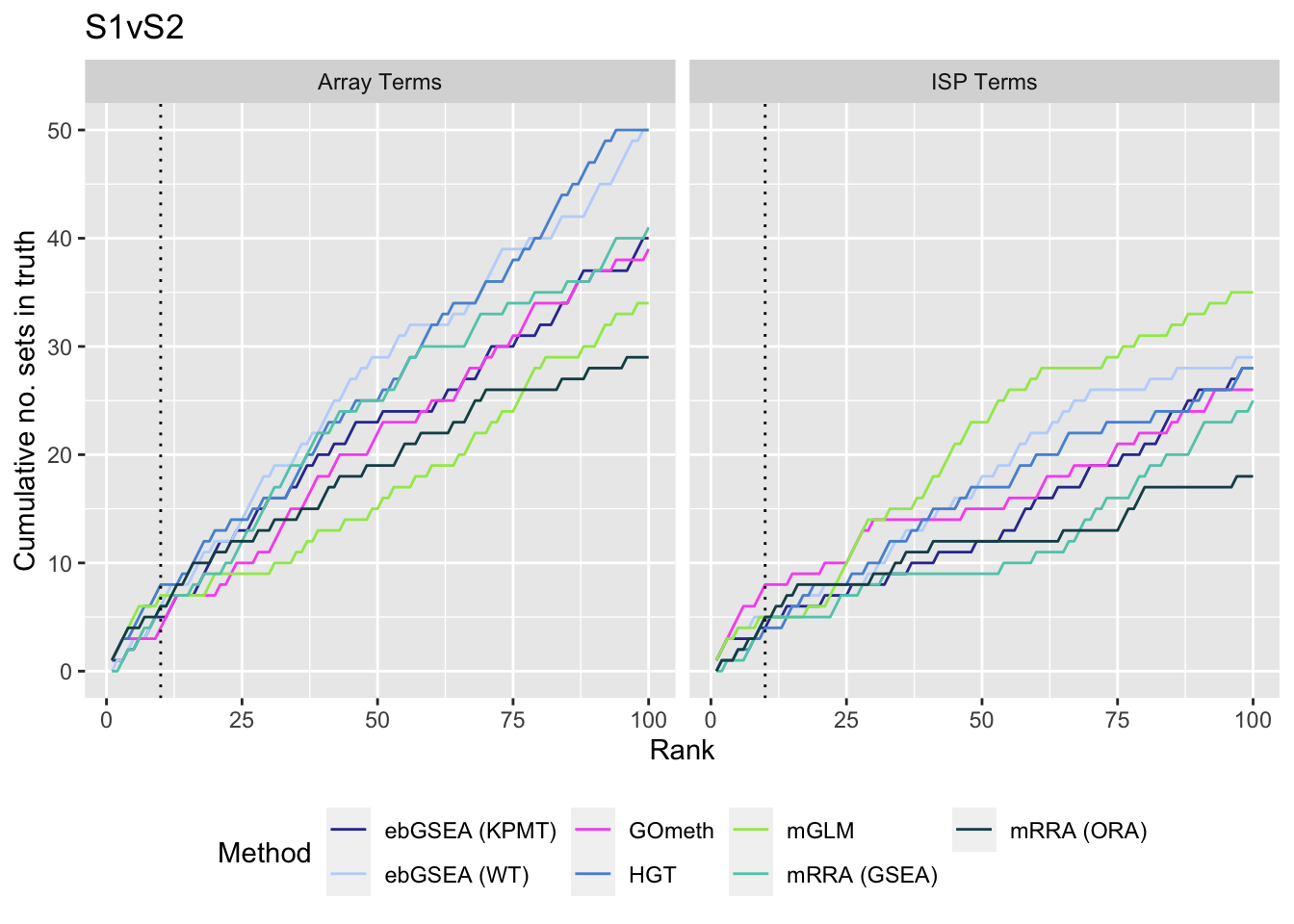Version Author Date
4c57176 JovMaksimovic 2021-04-13
17cc1c9 JovMaksimovic 2021-04-06
9029ba4 Jovana Maksimovic 2021-04-02

Save figure for use in manuscript.

fig <- here("output/figures/SFig-9A.rds")
saveRDS(p[], fig, compress = FALSE)

Examine what the top 10 ranked gene sets are and how many genes they contain, for each method and comparison.

terms <- missMethyl:::.getKEGG()$idTable nGenes <- rownames_to_column(data.frame(n = sapply(missMethyl:::.getKEGG()$idList,
length)),
var = "ID")

dat %>% filter(set == "KEGG") %>%
filter(sub %in% c("n","p1")) %>%
mutate(method = unname(dict[method])) %>%
arrange(contrast, method, pvalue) %>%
group_by(contrast, method) %>%
mutate(FDR = p.adjust(pvalue, method = "BH")) %>%
mutate(rank = 1:n()) %>%
filter(rank <= 10) %>%
inner_join(terms, by = c("ID" = "PathwayID")) %>%
inner_join(nGenes) -> sub
Joining, by = "ID"
p <- vector("list", length(unique(sub$contrast))) for(i in 1:length(p)){ cont <- sort(unique(sub$contrast))[i]
sub %>% filter(contrast == cont) %>%
arrange(method, -rank) %>%
ungroup() %>%
mutate(idx = as.factor(1:n())) -> tmp

setLabs <- substr(tmp$Description, 1, 40) names(setLabs) <- tmp$idx

tmp %>% mutate(rna = ID %in% topKEGG$PID[topKEGG$contrast %in% cont],
isp = ID %in% immuneKEGG$PID, both = rna + isp, col = ifelse(both == 2, "Both", ifelse(both == 1 & rna == 1, "Array", ifelse(both == 1 & isp == 1, "ISP", "Neither")))) %>% mutate(col = factor(col, levels = c("Both", "ISP", "Array", "Neither"))) -> tmp p[[i]] <- ggplot(tmp, aes(x = -log10(FDR), y = idx, colour = col)) + geom_point(aes(size = n), alpha = 0.7) + scale_size(limits = c(min(sub$n), max(sub$n))) + facet_wrap(vars(method), ncol = 2, scales = "free") + scale_y_discrete(labels = setLabs) + scale_colour_manual(values = truthPal) + labs(y = "", size = "No. genes", colour = "In truth set") + theme(axis.text.y = element_text(size = 6), axis.text.x = element_text(size = 6), legend.box = "horizontal", legend.margin = margin(0, 0, 0, 0, unit = "lines"), panel.spacing.x = unit(1, "lines")) + coord_cartesian(xlim = c(-log10(0.99), -log10(10^-80))) + geom_vline(xintercept = -log10(0.05), linetype = "dashed") + ggtitle(cont) } shift_legend(p[], plot = TRUE, pos = "left")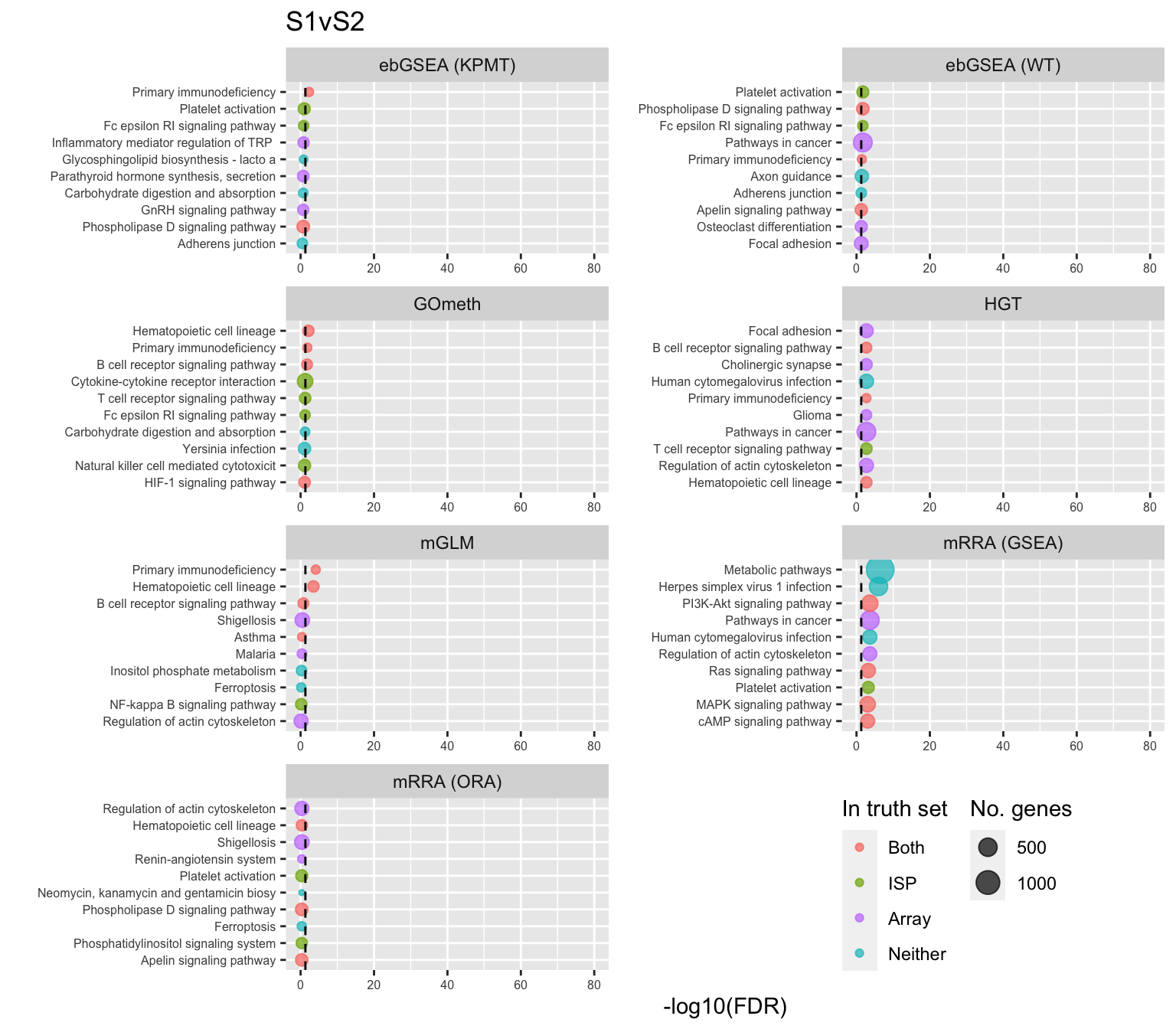Version Author Date 0f8d628 JovMaksimovic 2021-04-20 4c57176 JovMaksimovic 2021-04-13 Save figure for use in manuscript. fig <- here("output/figures/SFig-9B.rds") saveRDS(shift_legend(p[] + theme(plot.title = element_blank()), pos = "left"), fig, compress = FALSE) P-value histograms for the different methods for all contrasts on KEGG pathways. dat %>% filter(set == "KEGG") %>% filter(sub %in% c("n","p1")) %>% mutate(method = unname(dict[method])) -> subDat ggplot(subDat, aes(pvalue, fill = method)) + geom_histogram(binwidth = 0.025) + facet_wrap(vars(method), ncol = 2, nrow = 4) + theme(legend.position = "bottom") + labs(x = "P-value", y = "Frequency", fill = "Method") + scale_fill_manual(values = methodCols)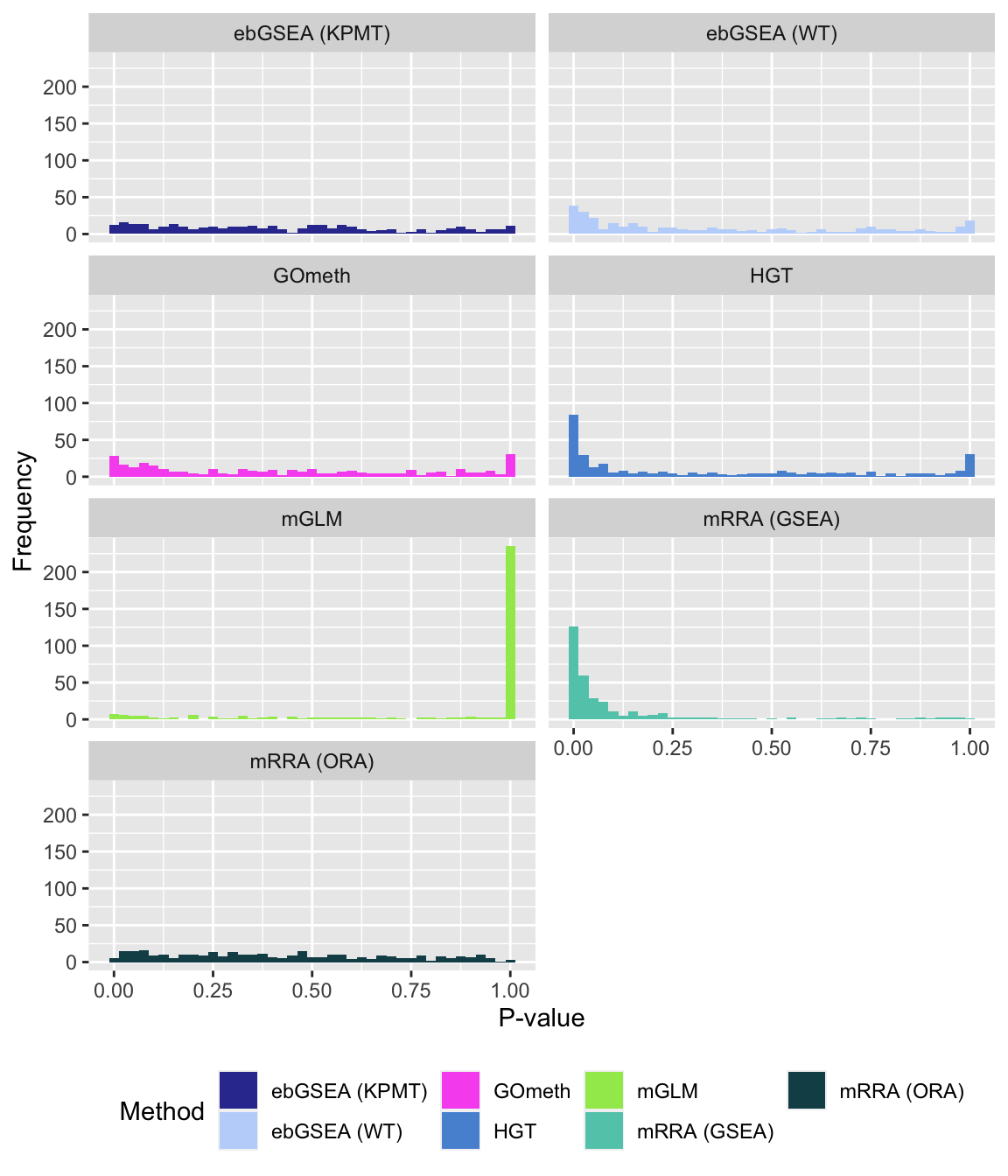Version Author Date 4c57176 JovMaksimovic 2021-04-13 17cc1c9 JovMaksimovic 2021-04-06 9029ba4 Jovana Maksimovic 2021-04-02 #### Compare GOmeth results using different DM CpG significance cutoffs As the results of GOmeth depend on the list of significant CpGs provided as input to the function, we explored the effect of selecting "significant" CpGs in different ways on the gene set testing performance of GOmeth. dat %>% filter(set == "KEGG") %>% filter(grepl("mmethyl", method)) %>% mutate(method = unname(dict[method])) %>% arrange(contrast, method, pvalue) %>% group_by(contrast, method, sub) %>% mutate(csum = cumsum(ID %in% immuneKEGG$PID)) %>%
mutate(rank = 1:n()) %>%
mutate(cut = ifelse(sub == "c1", "Top 5000",
ifelse(sub == "c2", "Top 10000",
ifelse(sub == "p1", "FDR < 0.01", "FDR < 0.05")))) %>%
filter(rank <= 100) -> sub

p <- ggplot(sub, aes(x = rank, y = csum, colour = cut)) +
geom_line() +
facet_wrap(vars(method), ncol=2, nrow = 3, scales = "free") +
geom_vline(xintercept = 10, linetype = "dotted") +
labs(colour = "Sig. select", x = "Rank", y = "Cumulative no. immune sets") +
theme(legend.position = "bottom")
p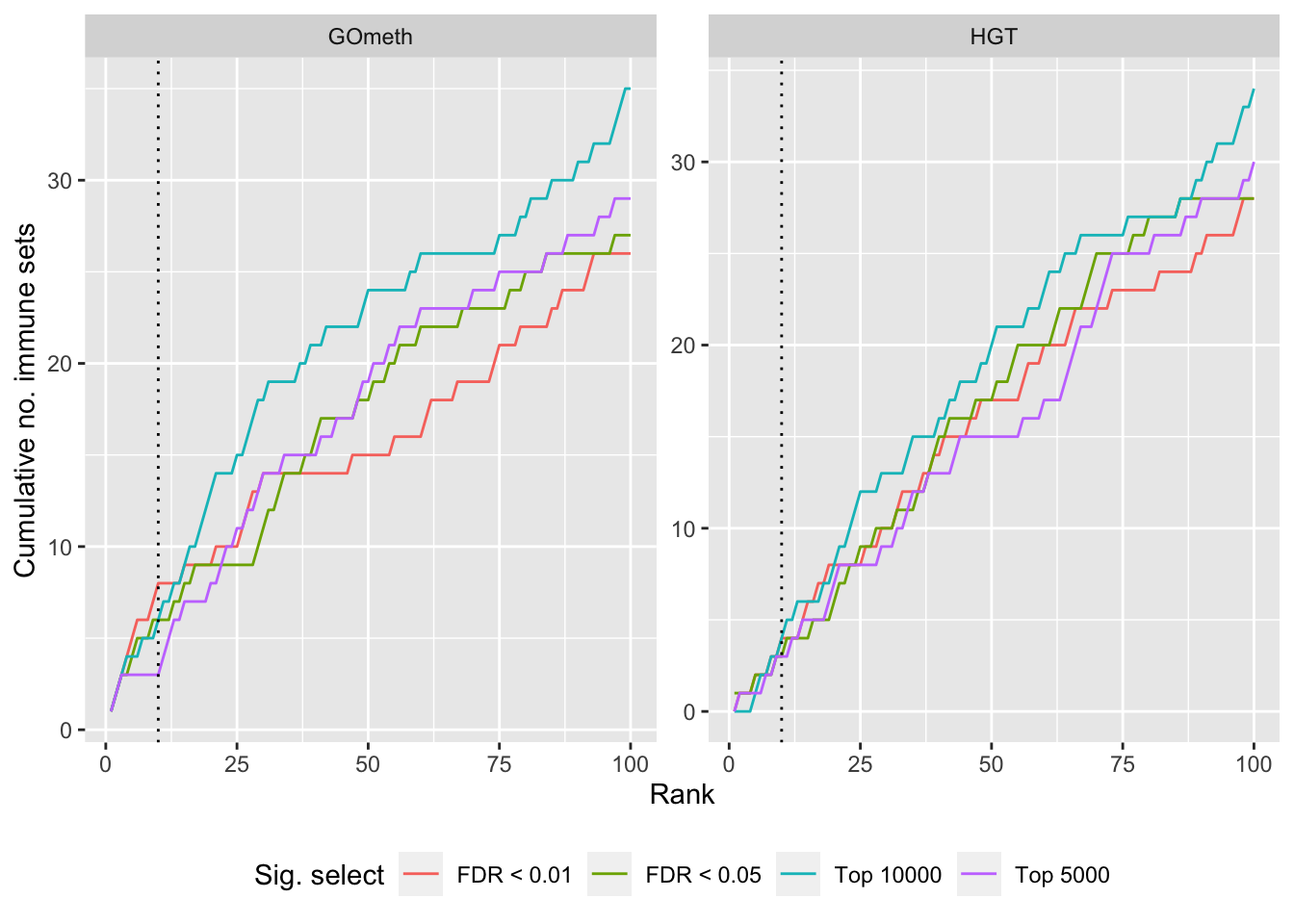Version Author Date
4c57176 JovMaksimovic 2021-04-13
17cc1c9 JovMaksimovic 2021-04-06
9029ba4 Jovana Maksimovic 2021-04-02
dat %>% filter(set == "KEGG") %>%
filter(grepl("mmethyl", method)) %>%
mutate(method = unname(dict[method])) %>%
arrange(contrast, method, pvalue) %>%
group_by(contrast, method, sub) %>%
mutate(csum = cumsum(ID %in% topKEGG$PID[topKEGG$contrast %in% contrast])) %>%
mutate(rank = 1:n()) %>%
mutate(cut = ifelse(sub == "c1", "Top 5000",
ifelse(sub == "c2", "Top 10000",
ifelse(sub == "p1", "FDR < 0.01", "FDR < 0.05")))) %>%
filter(rank <= 100) -> sub

p <- ggplot(sub, aes(x = rank, y = csum, colour = cut)) +
geom_line() +
facet_wrap(vars(method), ncol=2, nrow = 3, scales = "free") +
geom_vline(xintercept = 10, linetype = "dotted") +
labs(colour = "Sig. select", x = "Rank",
y = glue("Cumulative no. Array sets")) +
theme(legend.position = "bottom")
p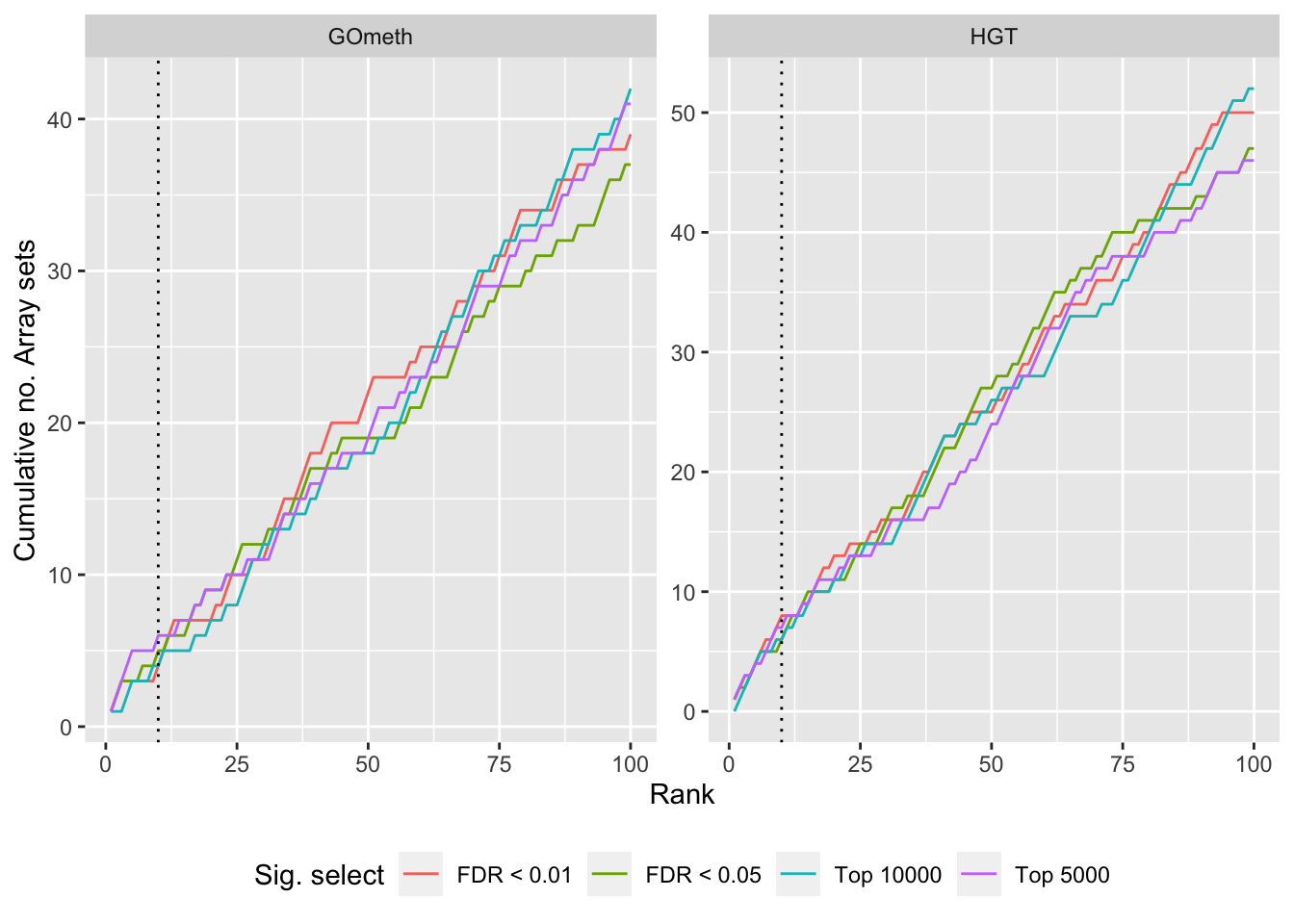Version Author Date
4c57176 JovMaksimovic 2021-04-13
17cc1c9 JovMaksimovic 2021-04-06

Compare methods by testing the in-built database of Broad Institute gene sets provided with the ChAMP. Using the top 100 ranked gene sets as identified by gsaseq analysis of the corresponding B-cell development stages data as "truth".

rnaseqBROAD <- readRDS(here("data/cache-rnaseq/RNAseq-GSE45460-BROAD-GSA.rds"))
mutate(rank = 1:n()) %>%

p <- vector("list", length(unique(dat$contrast))) for(i in 1:length(unique(dat$contrast))){
cont <- sort(unique(dat$contrast))[i] dat %>% filter(set == "BROAD") %>% filter(sub %in% c("n","p1")) %>% filter(contrast == cont) %>% mutate(method = unname(dict[method])) %>% arrange(contrast, method, pvalue) %>% group_by(contrast, method) %>% mutate(csum = cumsum(ID %in% topBROAD$ID[topBROAD$contrast %in% contrast])) %>% mutate(rank = 1:n()) %>% filter(rank <= 100) -> sub p[[i]] <- ggplot(sub, aes(x = rank, y = csum, colour = method)) + geom_line() + geom_vline(xintercept = 10, linetype = "dotted") + labs(colour = "Method", x = "Rank", y = "Cumulative no. Array sets") + theme(legend.position = "bottom") + scale_color_manual(values = methodCols) + ggtitle(cont) } p[]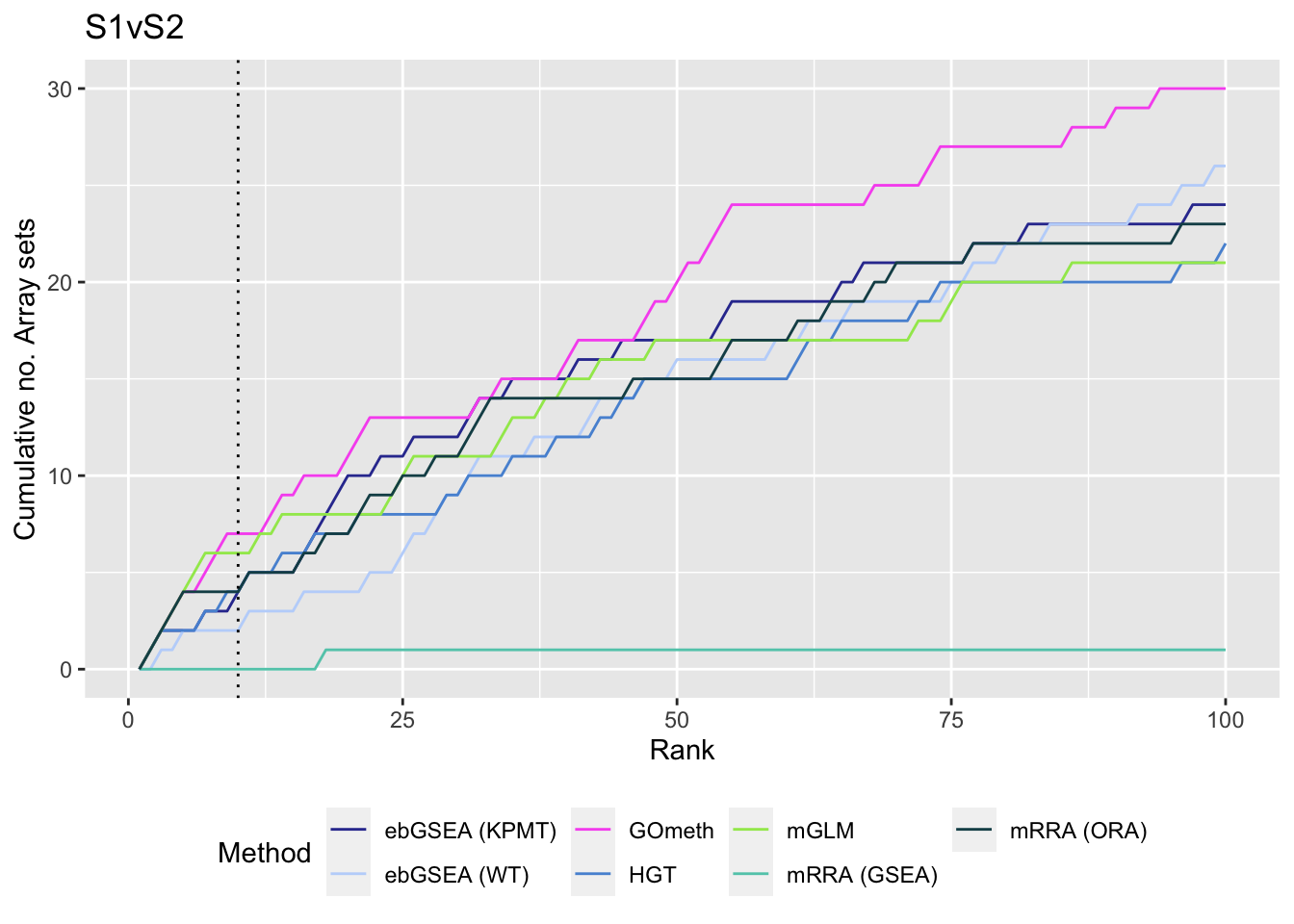Version Author Date 4c57176 JovMaksimovic 2021-04-13 17cc1c9 JovMaksimovic 2021-04-06 Examine what the top 10 ranked gene sets are and how many genes they contain, for each method and comparison. data(PathwayList) nGenes <- rownames_to_column(data.frame(n = sapply(PathwayList, length)), var = "ID") dat %>% filter(set == "BROAD") %>% filter(sub %in% c("n","p1")) %>% mutate(method = unname(dict[method])) %>% arrange(contrast, method, pvalue) %>% group_by(contrast, method) %>% mutate(FDR = p.adjust(pvalue, method = "BH")) %>% mutate(rank = 1:n()) %>% filter(rank <= 10) %>% inner_join(nGenes) -> sub Joining, by = "ID" truthPal <- scales::hue_pal()(4)[3:4] names(truthPal) <- c("None", "Array") p <- vector("list", length(unique(sub$contrast)))
for(i in 1:length(p)){
cont <- sort(unique(sub$contrast))[i] sub %>% filter(contrast == cont) %>% arrange(method, -rank) %>% ungroup() %>% mutate(idx = as.factor(1:n())) -> tmp setLabs <- substr(tmp$ID, 1, 30)
names(setLabs) <- tmp$idx tmp %>% mutate(rna = ID %in% topBROAD$ID[topGOSets$contrast %in% cont], col = ifelse(rna == 1, "Array", "None")) %>% mutate(col = factor(col, levels = c("Array", "None"))) -> tmp p[[i]] <- ggplot(tmp, aes(x = -log10(FDR), y = idx, colour = col)) + geom_point(aes(size = n), alpha = 0.7) + scale_size(limits = c(min(sub$n), max(sub$n))) + facet_wrap(vars(method), ncol = 2, scales = "free") + scale_y_discrete(labels = setLabs) + scale_color_manual(values = truthPal) + labs(y = "", size = "No. genes", colour = "In truth set") + theme(axis.text.y = element_text(size = 6), axis.text.x = element_text(size = 6), legend.box = "horizontal", legend.margin = margin(0, 0, 0, 0, unit = "lines"), panel.spacing.x = unit(1, "lines")) + coord_cartesian(xlim = c(-log10(0.99), -log10(10^-100))) + geom_vline(xintercept = -log10(0.05), linetype = "dashed") + ggtitle(cont) } shift_legend(p[], plot = TRUE, pos = "left")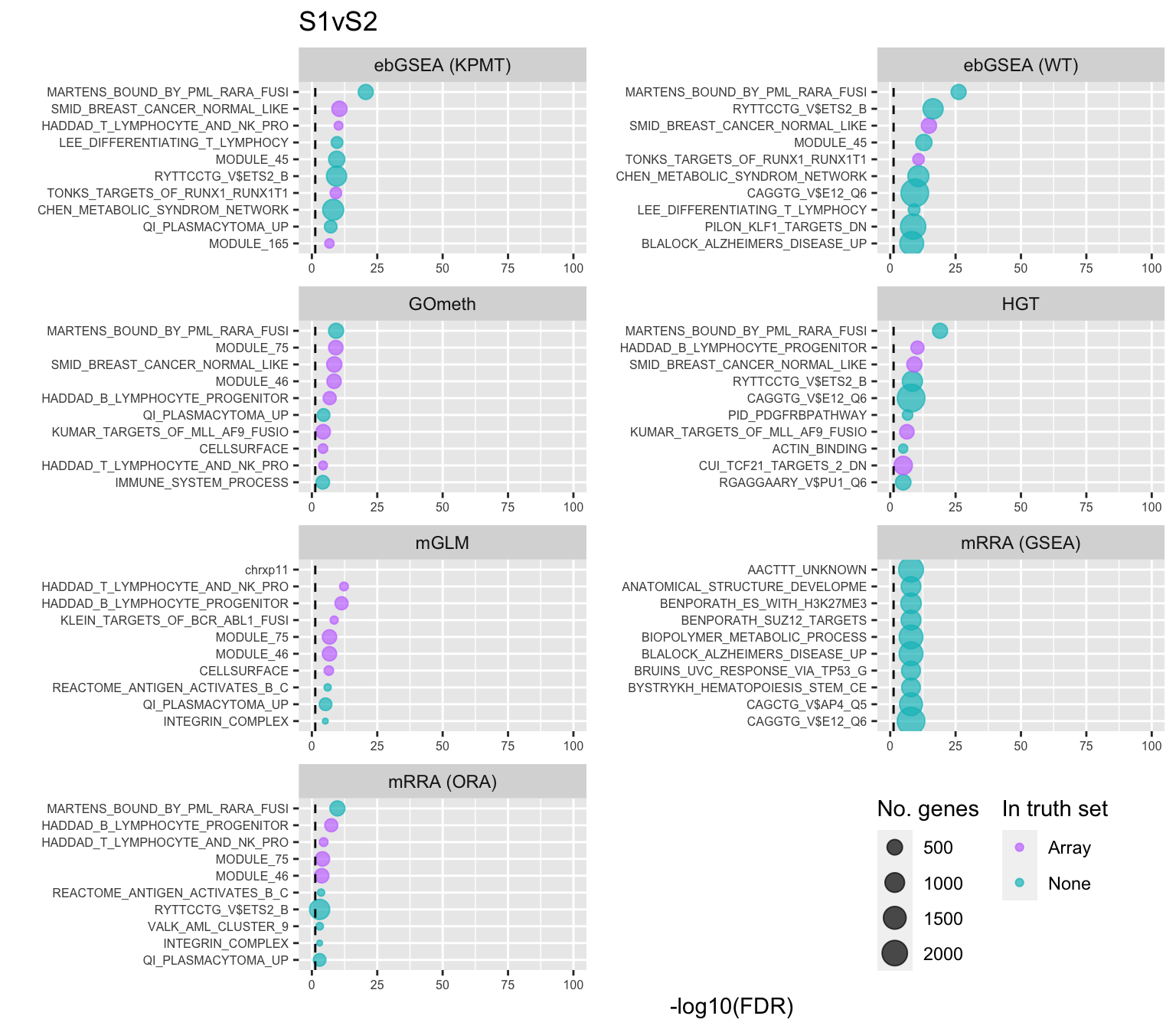Version Author Date 4c57176 JovMaksimovic 2021-04-13 P-value histograms for the different methods for all contrasts on BROAD gene sets. dat %>% filter(set == "BROAD") %>% filter(sub %in% c("n","p1")) %>% mutate(method = unname(dict[method])) -> subDat ggplot(subDat, aes(pvalue, fill = method)) + geom_histogram(binwidth = 0.025) + facet_wrap(vars(method), ncol = 2, nrow = 4) + theme(legend.position = "bottom") + labs(x = "P-value", y = "Frequency", fill = "Method") + scale_fill_manual(values = methodCols)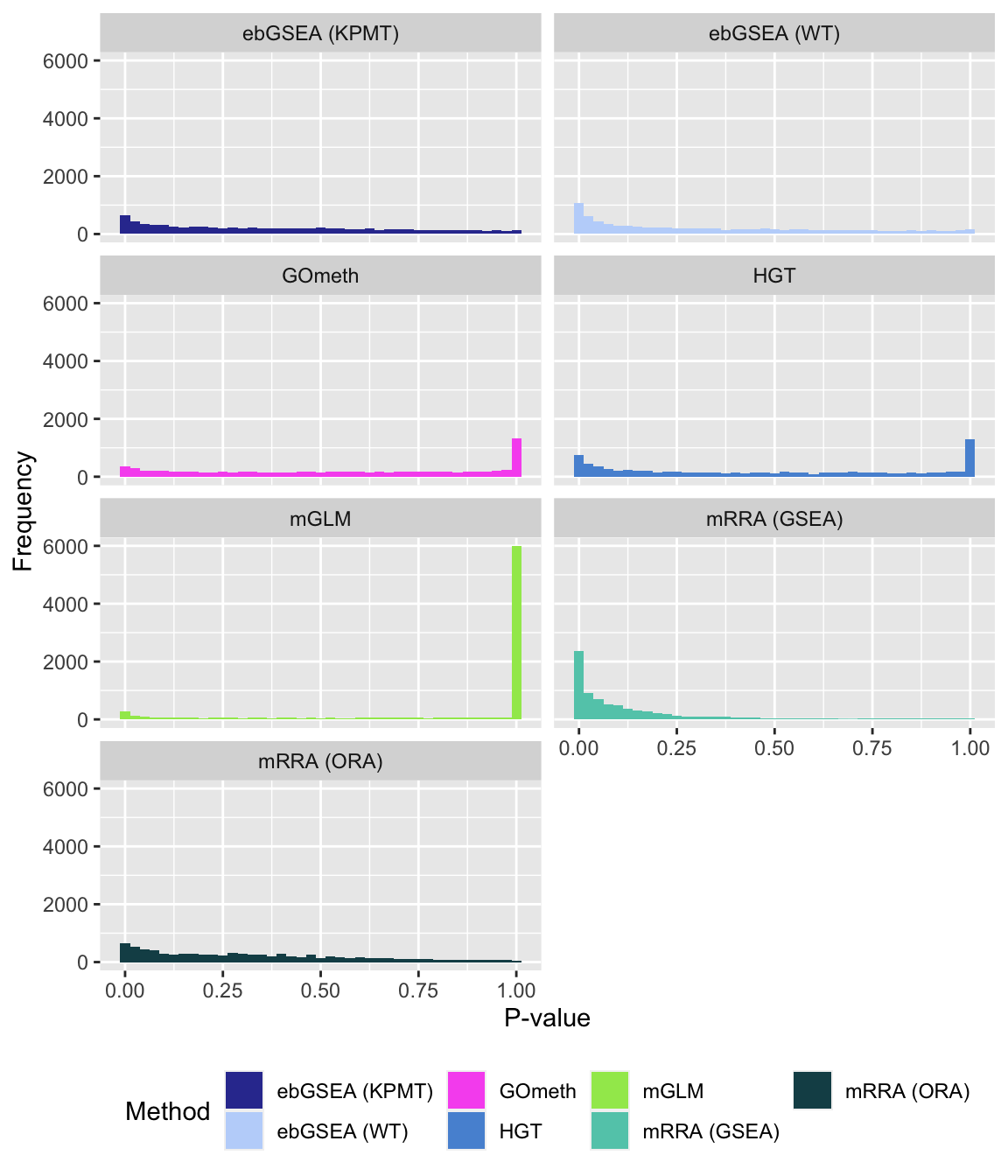Version Author Date 4c57176 JovMaksimovic 2021-04-13 17cc1c9 JovMaksimovic 2021-04-06 9029ba4 Jovana Maksimovic 2021-04-02 #### Compare GOmeth results using different DM CpG significance cutoffs As the results of GOmeth depend on the list of significant CpGs provided as input to the function, we explored the effect of selecting "significant" CpGs in different ways on the gene set testing performance of GOmeth. dat %>% filter(set == "BROAD") %>% filter(grepl("mmethyl", method)) %>% mutate(method = unname(dict[method])) %>% arrange(contrast, method, pvalue) %>% group_by(contrast, method, sub) %>% mutate(csum = cumsum(ID %in% topBROAD$ID)) %>%
mutate(rank = 1:n()) %>%
mutate(cut = ifelse(sub == "c1", "Top 5000",
ifelse(sub == "c2", "Top 10000",
ifelse(sub == "p1", "FDR < 0.01", "FDR < 0.05")))) %>%
filter(rank <= 100) -> sub

p <- ggplot(sub, aes(x = rank, y = csum, colour = cut)) +
geom_line() +
facet_wrap(vars(method), ncol=2, nrow = 3, scales = "free") +
geom_vline(xintercept = 10, linetype = "dotted") +
labs(colour = "Sig. select", x = "Rank", y = "Cumulative no. Array sets") +
theme(legend.position = "bottom")
p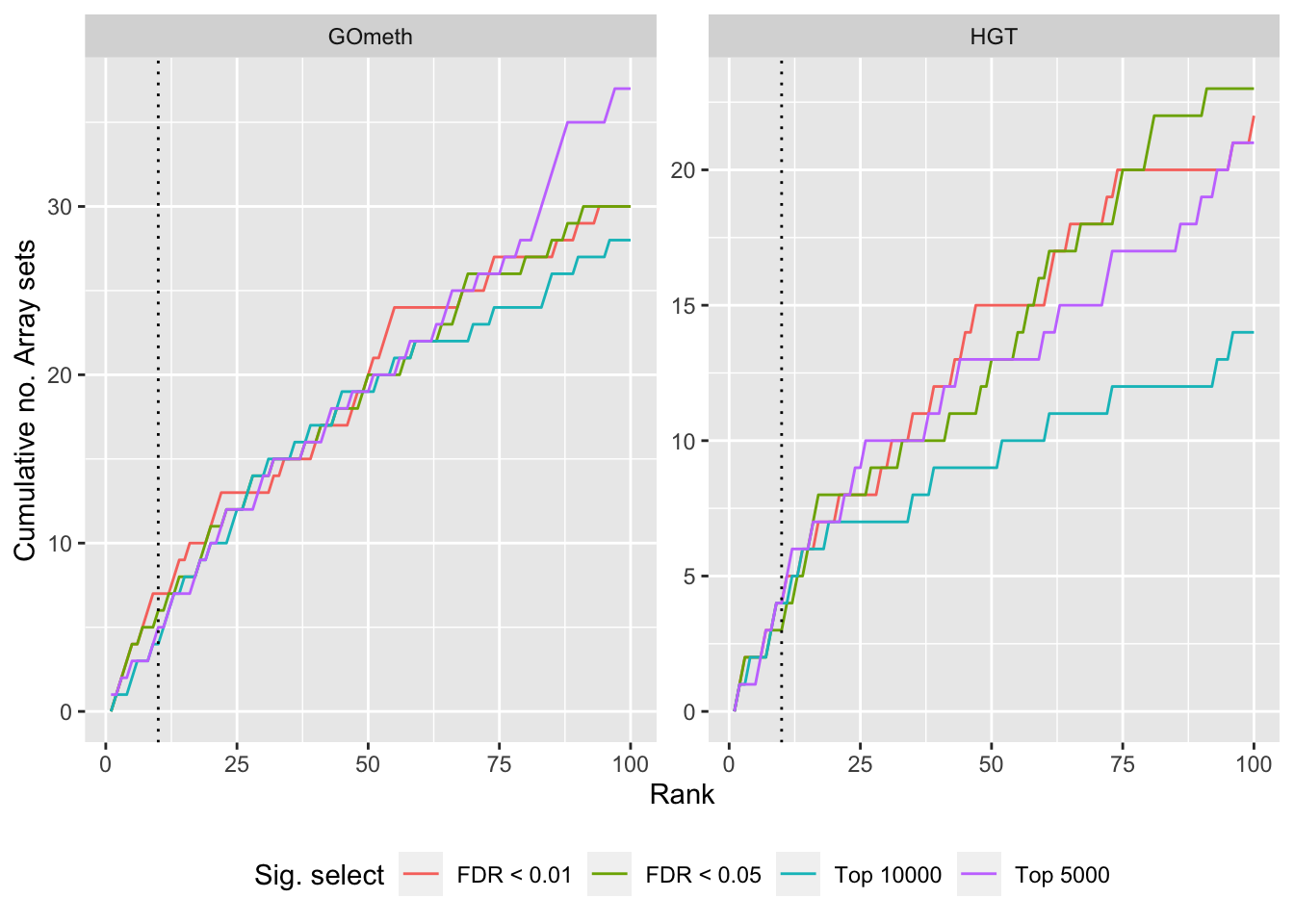Version Author Date
4c57176 JovMaksimovic 2021-04-13
17cc1c9 JovMaksimovic 2021-04-06
9029ba4 Jovana Maksimovic 2021-04-02

sessionInfo()
R version 4.0.3 (2020-10-10)
Platform: x86_64-apple-darwin17.0 (64-bit)
Running under: macOS Mojave 10.14.6

Matrix products: default
BLAS:   /Library/Frameworks/R.framework/Versions/4.0/Resources/lib/libRblas.dylib
LAPACK: /Library/Frameworks/R.framework/Versions/4.0/Resources/lib/libRlapack.dylib

locale:
 en_AU.UTF-8/en_AU.UTF-8/en_AU.UTF-8/C/en_AU.UTF-8/en_AU.UTF-8

attached base packages:
 grid      stats4    parallel  stats     graphics  grDevices utils
 datasets  methods   base

other attached packages:
 lemon_0.4.5
 ChAMPdata_2.22.0
 patchwork_1.1.1
 rbin_0.2.0
 forcats_0.5.1
 stringr_1.4.0
 dplyr_1.0.5
 purrr_0.3.4
 tidyr_1.1.3
 tibble_3.1.0
 tidyverse_1.3.0
 glue_1.4.2
 ggplot2_3.3.3
 missMethyl_1.24.0
 IlluminaHumanMethylationEPICanno.ilm10b4.hg19_0.6.0
 IlluminaHumanMethylation450kanno.ilmn12.hg19_0.6.0
 gridExtra_2.3
 reshape2_1.4.4
 BiocParallel_1.24.1
 limma_3.46.0
 paletteer_1.3.0
 minfi_1.36.0
 bumphunter_1.32.0
 locfit_1.5-9.4
 iterators_1.0.13
 foreach_1.5.1
 Biostrings_2.58.0
 XVector_0.30.0
 SummarizedExperiment_1.20.0
 Biobase_2.50.0
 MatrixGenerics_1.2.1
 matrixStats_0.58.0
 GenomicRanges_1.42.0
 GenomeInfoDb_1.26.7
 IRanges_2.24.1
 S4Vectors_0.28.1
 BiocGenerics_0.36.0
 here_1.0.1
 workflowr_1.6.2

loaded via a namespace (and not attached):
 BiocFileCache_1.14.0      plyr_1.8.6
 splines_4.0.3             digest_0.6.27
 htmltools_0.5.1.1         GO.db_3.12.1
 fansi_0.4.2               magrittr_2.0.1
 memoise_2.0.0             annotate_1.68.0
 siggenes_1.64.0           prettyunits_1.1.1
 colorspace_2.0-0          rvest_1.0.0
 blob_1.2.1                rappdirs_0.3.3
 haven_2.3.1               xfun_0.22
 crayon_1.4.1              RCurl_1.98-1.3
 jsonlite_1.7.2            genefilter_1.72.1
 GEOquery_2.58.0           survival_3.2-10
 gtable_0.3.0              zlibbioc_1.36.0
 DelayedArray_0.16.3       Rhdf5lib_1.12.1
 HDF5Array_1.18.1          scales_1.1.1
 DBI_1.1.1                 rngtools_1.5
 Rcpp_1.0.6                xtable_1.8-4
 progress_1.2.2            bit_4.0.4
 mclust_5.4.7              preprocessCore_1.52.1
 httr_1.4.2                RColorBrewer_1.1-2
 ellipsis_0.3.1            farver_2.1.0
 pkgconfig_2.0.3           reshape_0.8.8
 XML_3.99-0.6              sass_0.3.1
 dbplyr_2.1.1              utf8_1.2.1
 labeling_0.4.2            tidyselect_1.1.0
 rlang_0.4.10              later_1.1.0.1
 AnnotationDbi_1.52.0      cellranger_1.1.0
 munsell_0.5.0             tools_4.0.3
 cachem_1.0.4              cli_2.4.0
 generics_0.1.0            RSQLite_2.2.5
 broom_0.7.6               evaluate_0.14
 fastmap_1.1.0             yaml_2.2.1
 rematch2_2.1.2            org.Hs.eg.db_3.12.0
 knitr_1.31                bit64_4.0.5
 fs_1.5.0                  beanplot_1.2
 scrime_1.3.5              nlme_3.1-152
 doRNG_1.8.2               sparseMatrixStats_1.2.1
 whisker_0.4               nor1mix_1.3-0
 xml2_1.3.2                biomaRt_2.46.3
 rstudioapi_0.13           compiler_4.0.3
 curl_4.3                  reprex_2.0.0
 statmod_1.4.35            bslib_0.2.4
 stringi_1.5.3             highr_0.8
 GenomicFeatures_1.42.3    lattice_0.20-41
 Matrix_1.3-2              multtest_2.46.0
 vctrs_0.3.7               pillar_1.5.1
 lifecycle_1.0.0           rhdf5filters_1.2.0
 jquerylib_0.1.3           cowplot_1.1.1
 data.table_1.14.0         bitops_1.0-6
 httpuv_1.5.5              rtracklayer_1.50.0
 R6_2.5.0                  promises_1.2.0.1
 codetools_0.2-18          MASS_7.3-53.1
 assertthat_0.2.1          rhdf5_2.34.0
 openssl_1.4.3             rprojroot_2.0.2
 withr_2.4.1               GenomicAlignments_1.26.0
 Rsamtools_2.6.0           GenomeInfoDbData_1.2.4
 mgcv_1.8-34               hms_1.0.0
 lubridate_1.7.10